• 保健液
•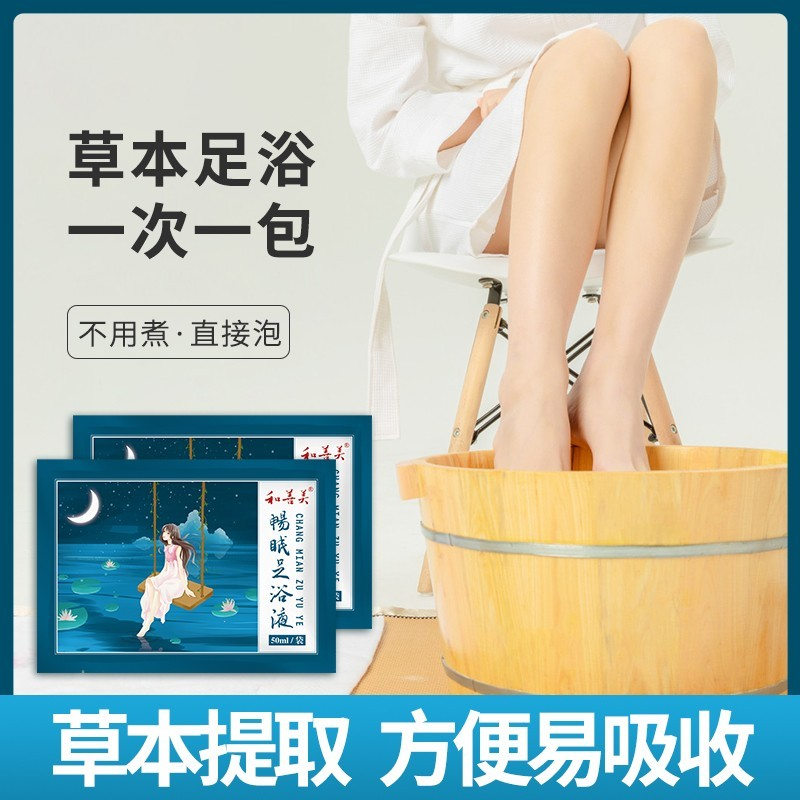畅眠足浴液
•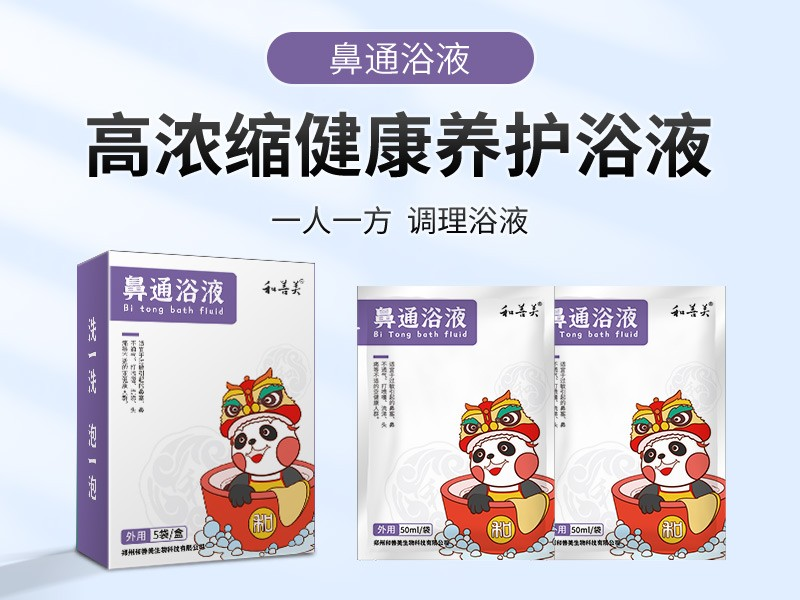鼻通浴液
•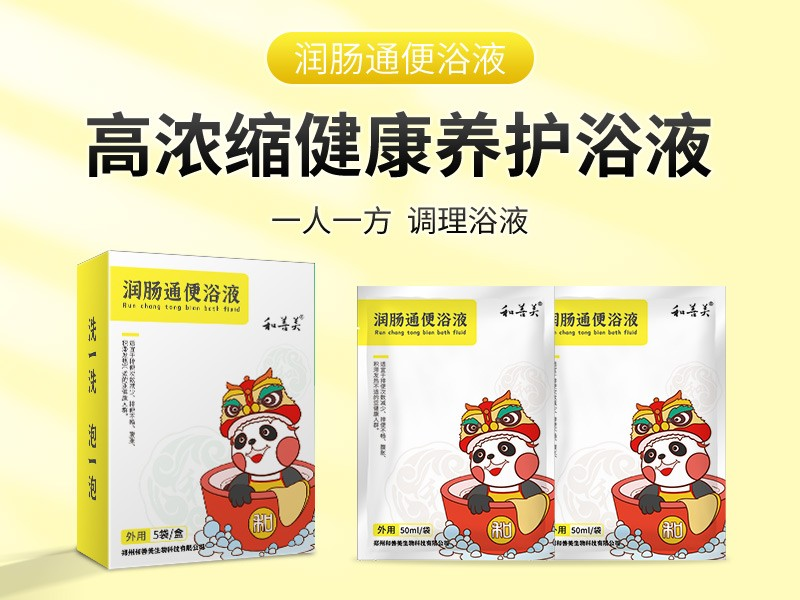润肠通便浴液
•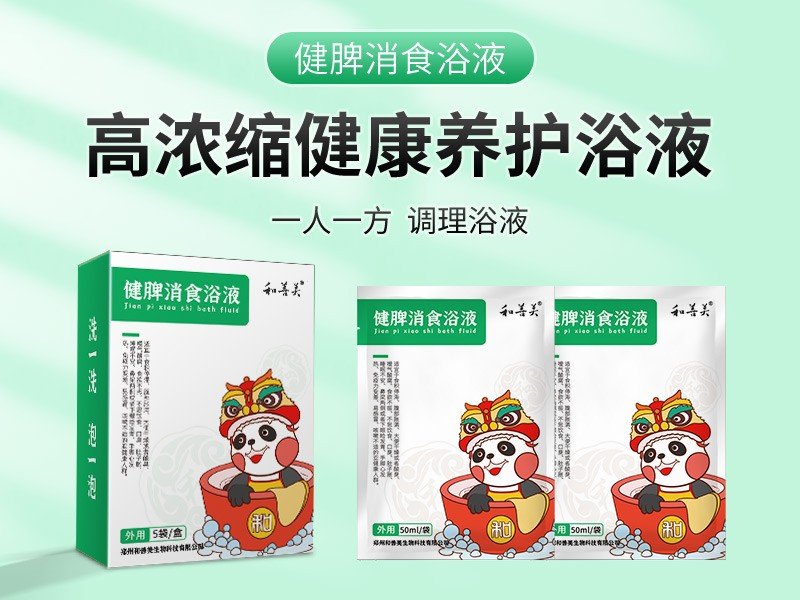健脾消食浴液
•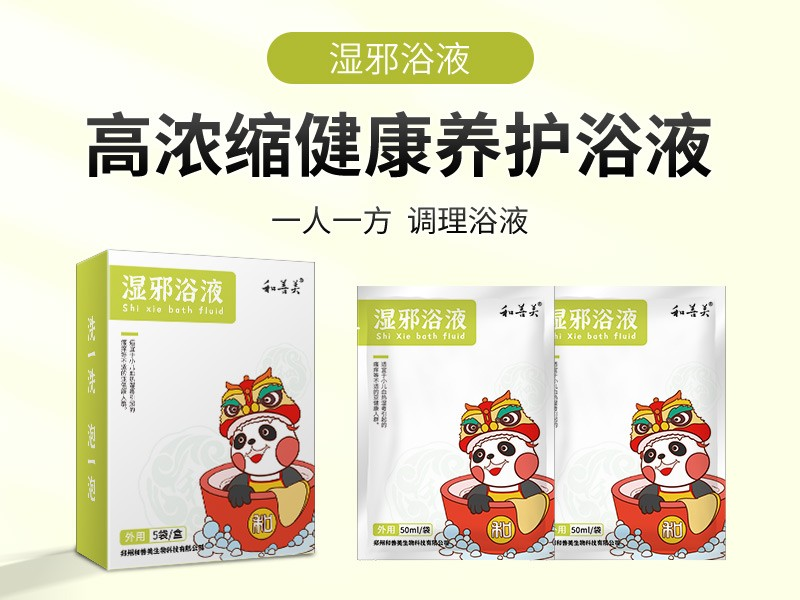湿邪浴液
•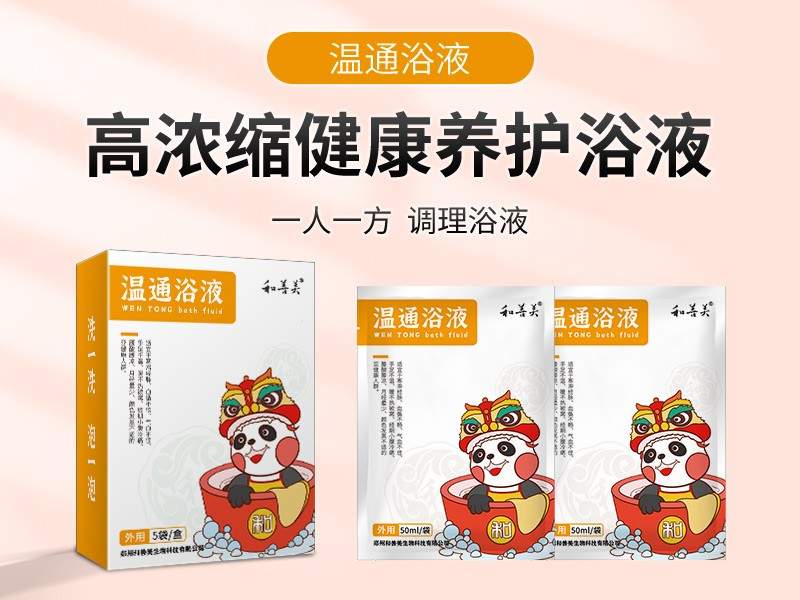温通浴液
•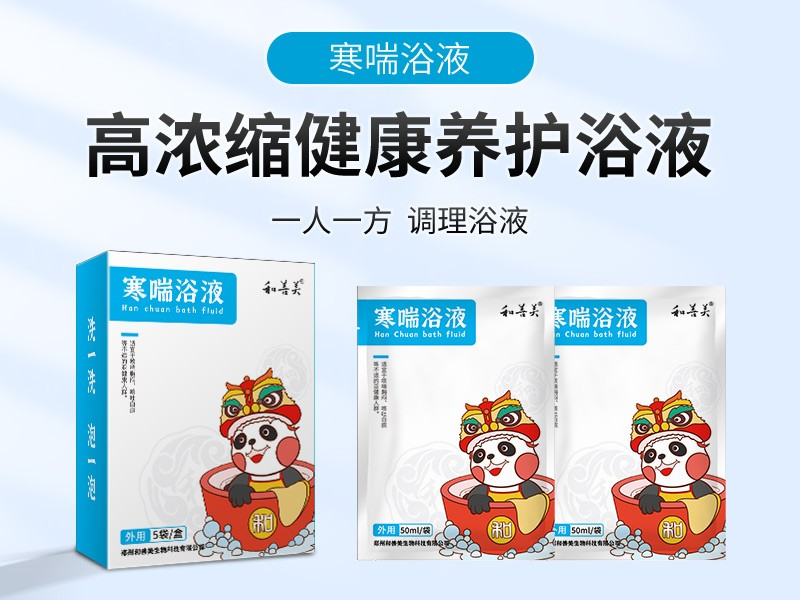寒喘浴液
•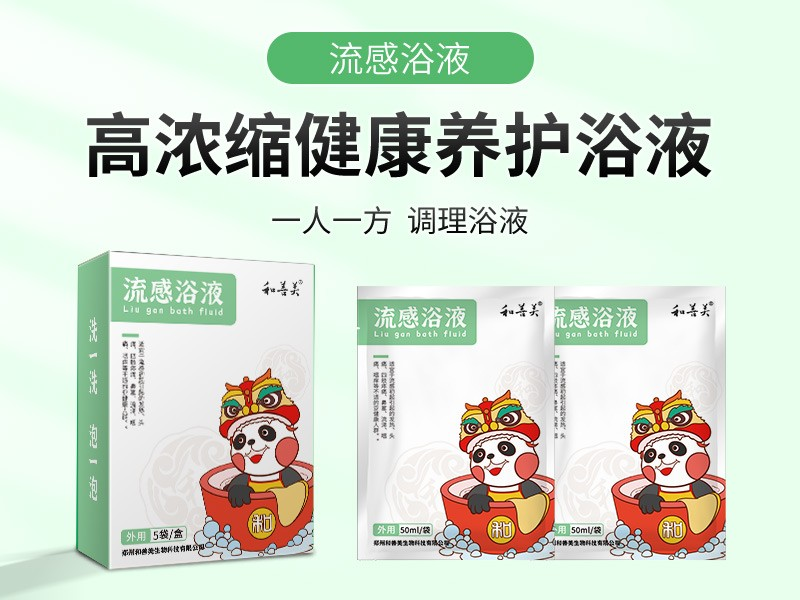流感浴液
•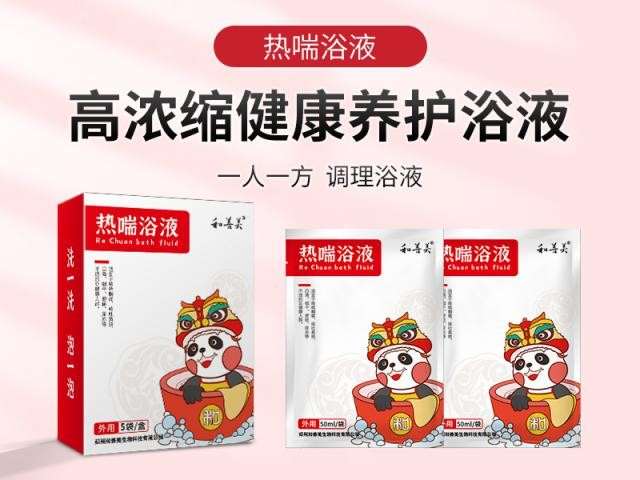热喘浴液
•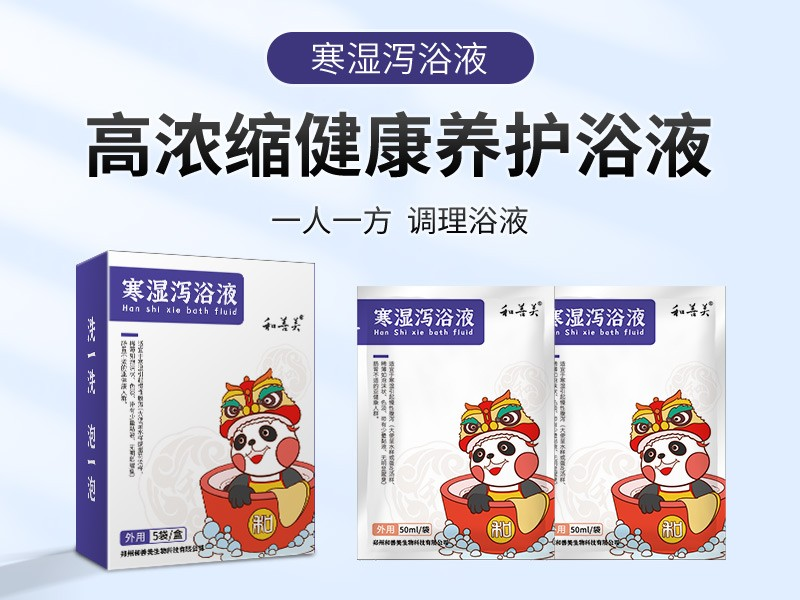寒湿泻浴液
•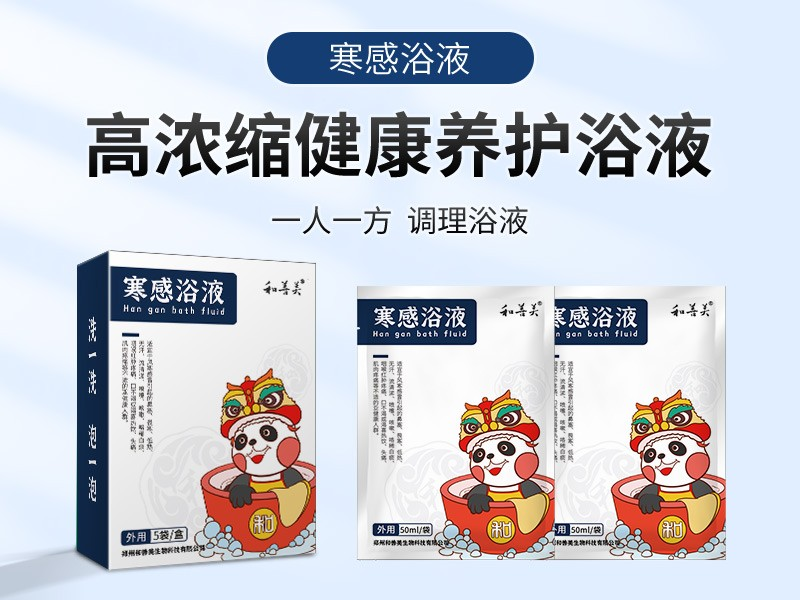寒感浴液
•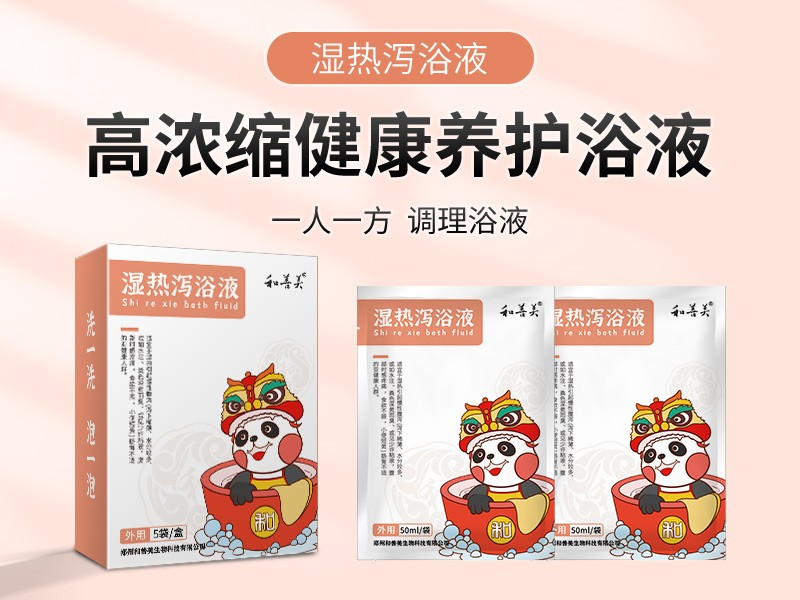湿热泻浴液
•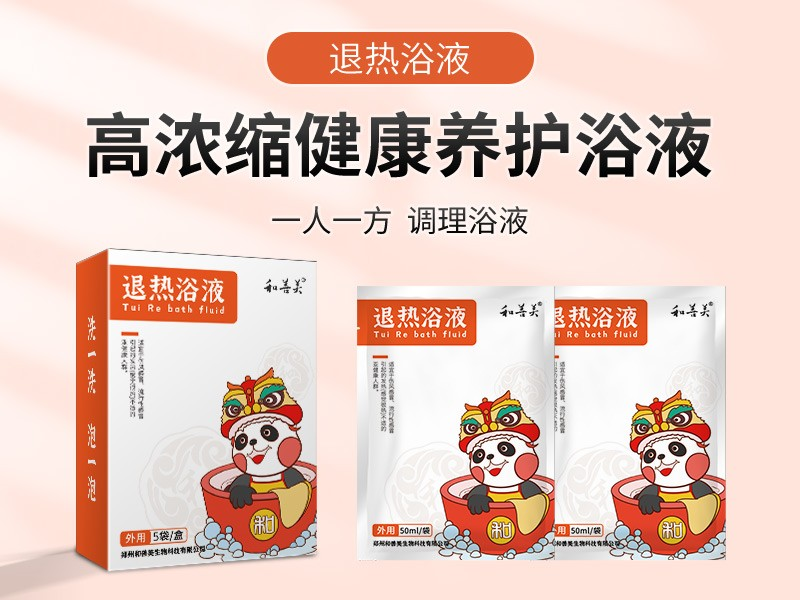退热浴液
•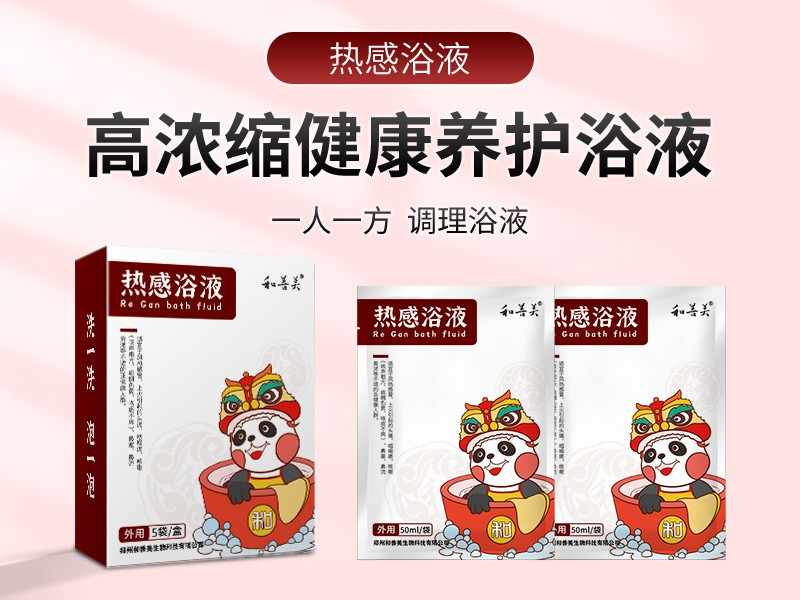热感浴液
•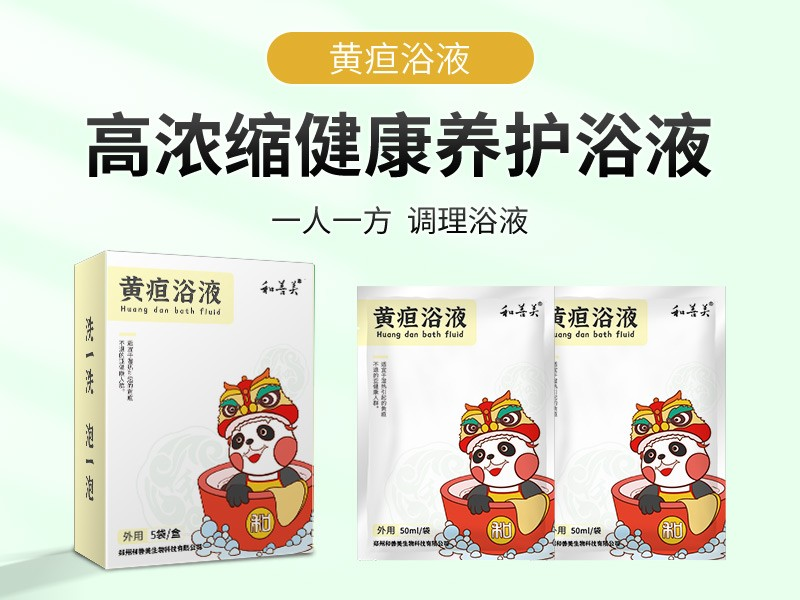黄疸浴液
• 穴位贴
•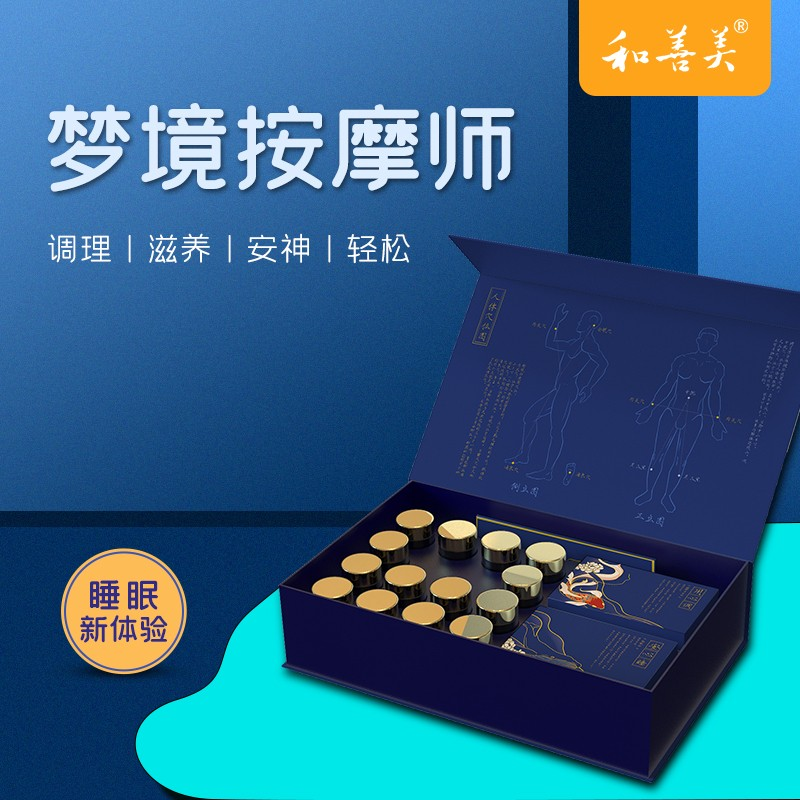梦境按摩师
•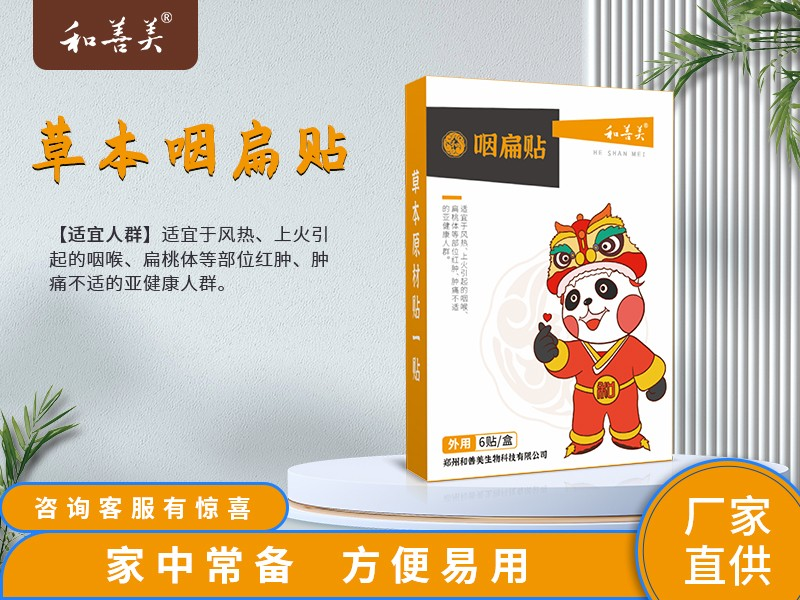咽扁贴
•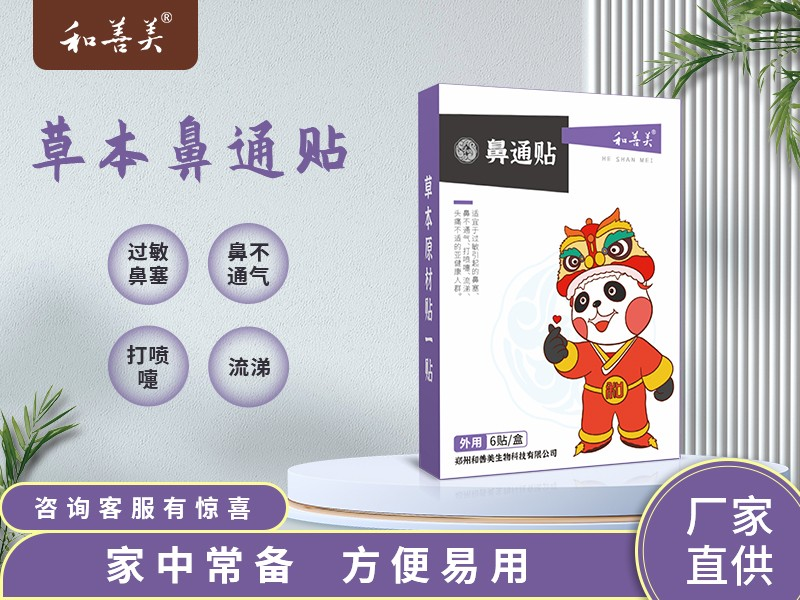鼻通贴
•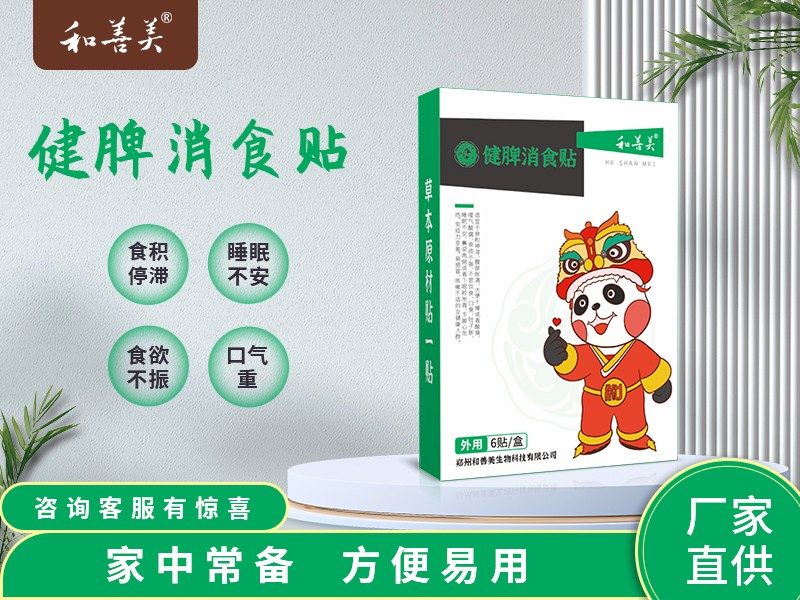健脾消食贴
•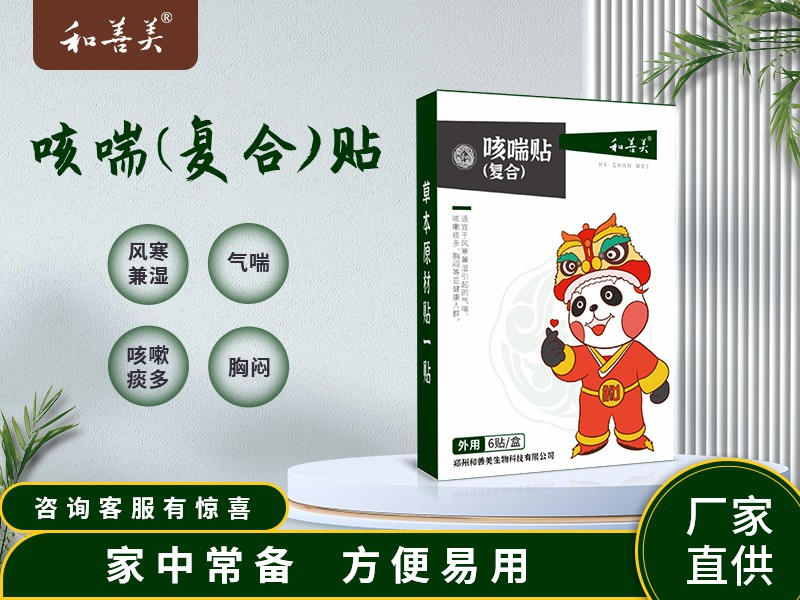咳喘贴(复合)
•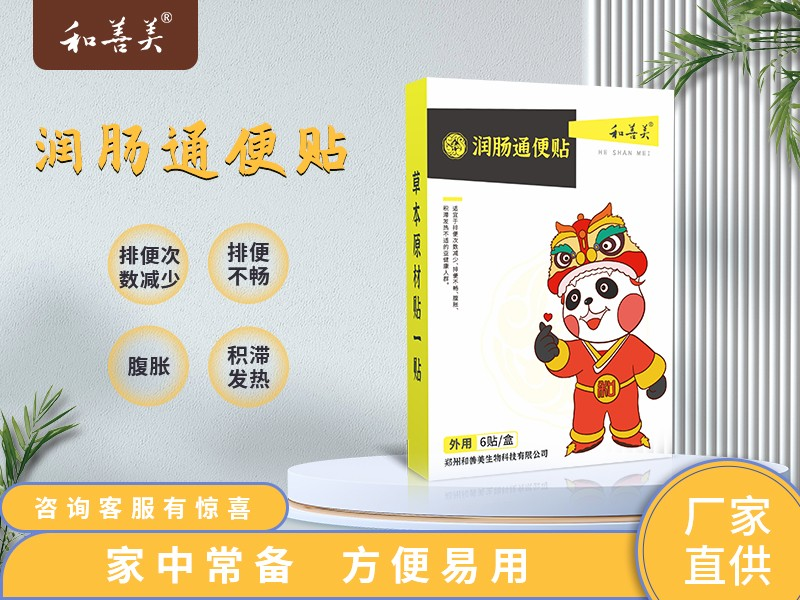润肠通便贴
•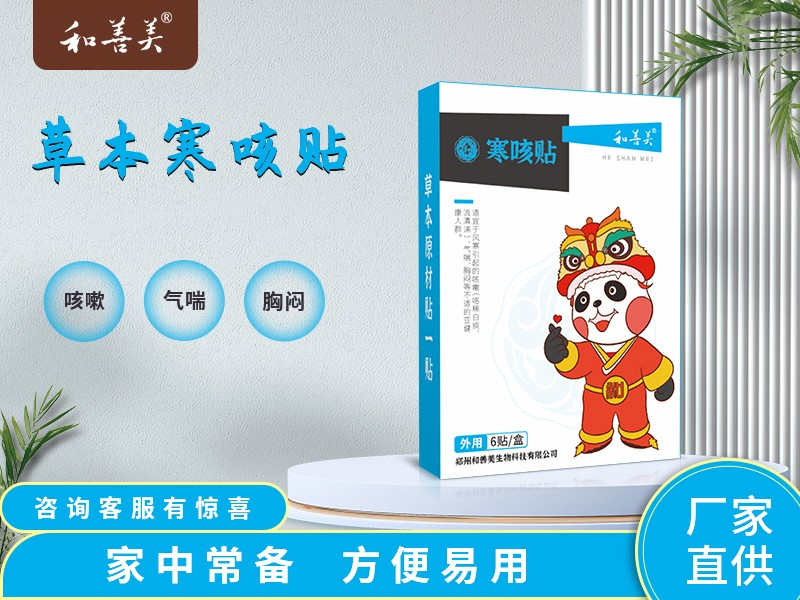寒咳贴
•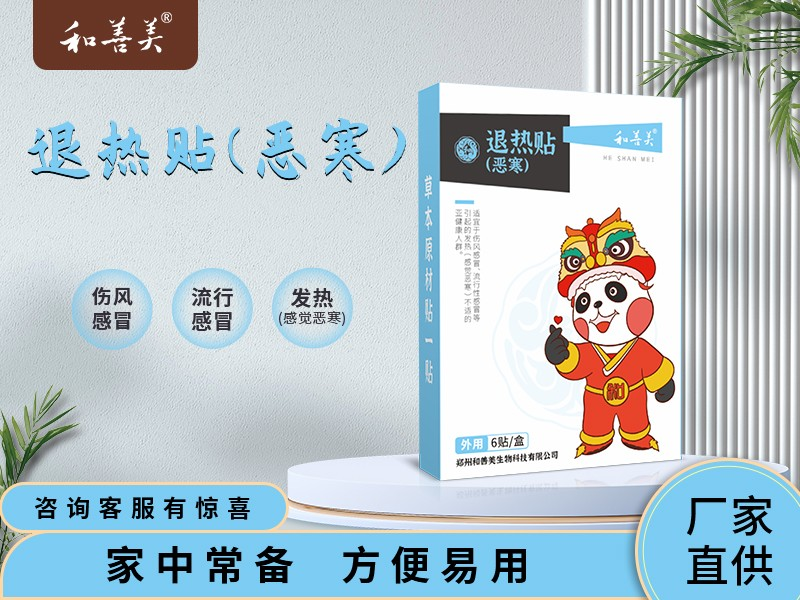退热贴(恶寒)
•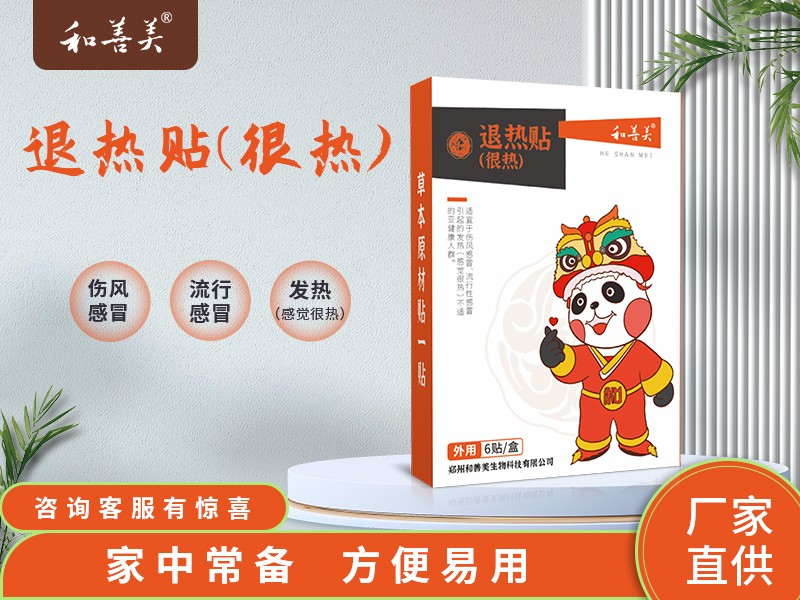退热贴（很热）
•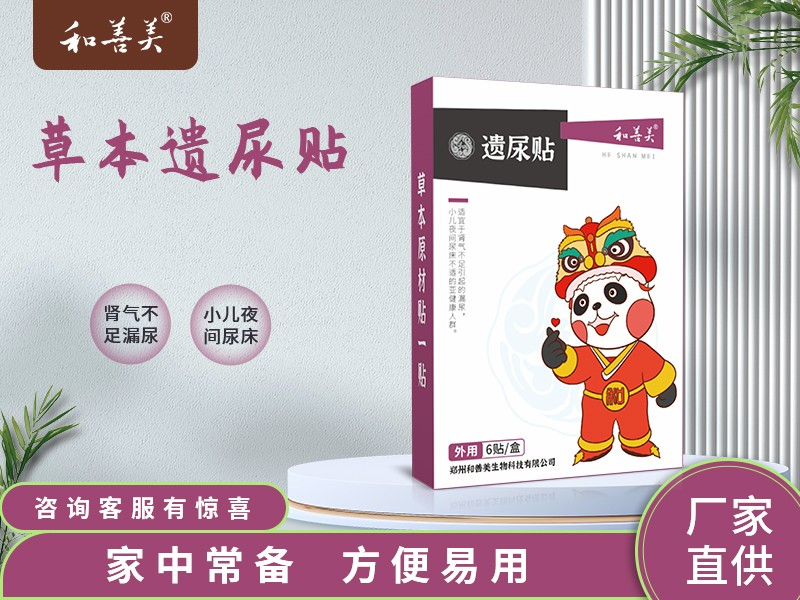遗尿贴
•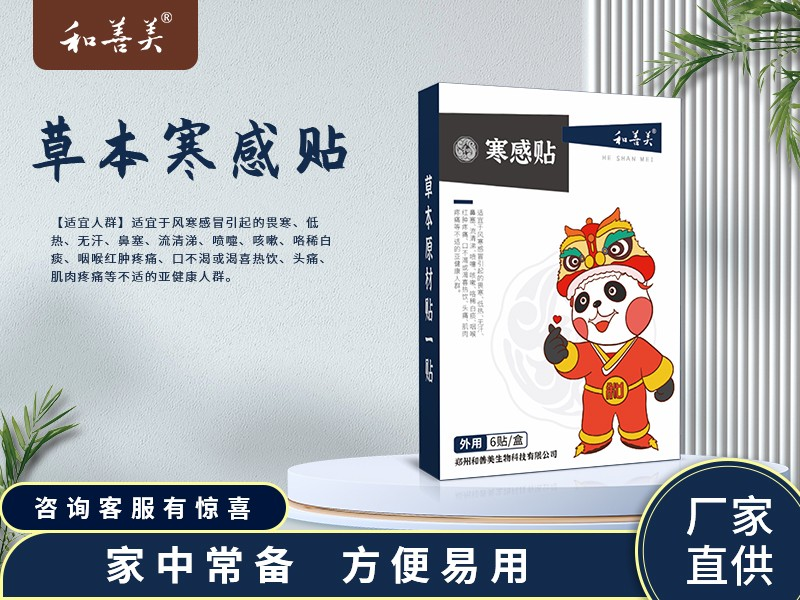寒感贴
•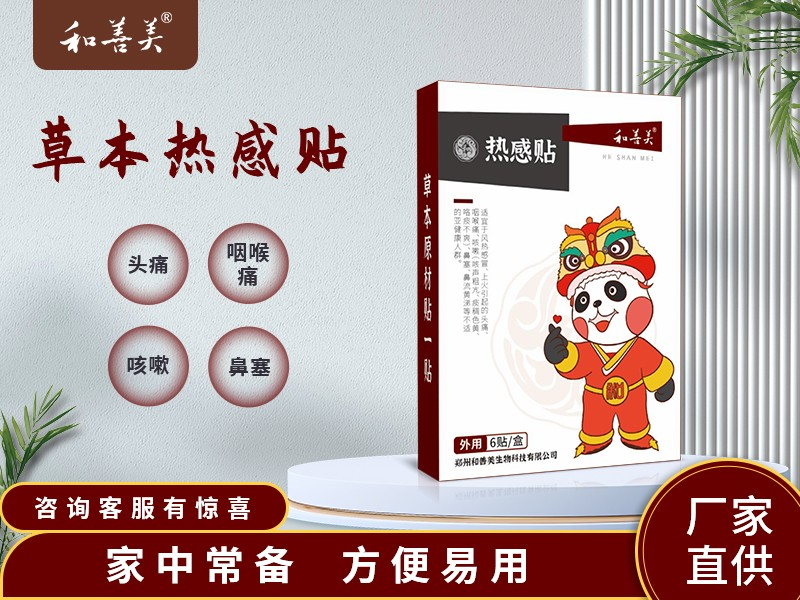热感贴
•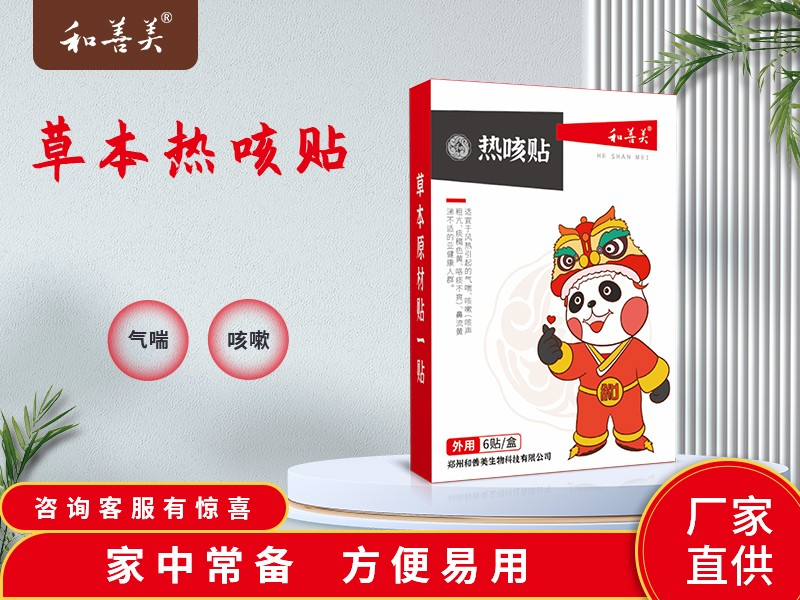热咳贴
•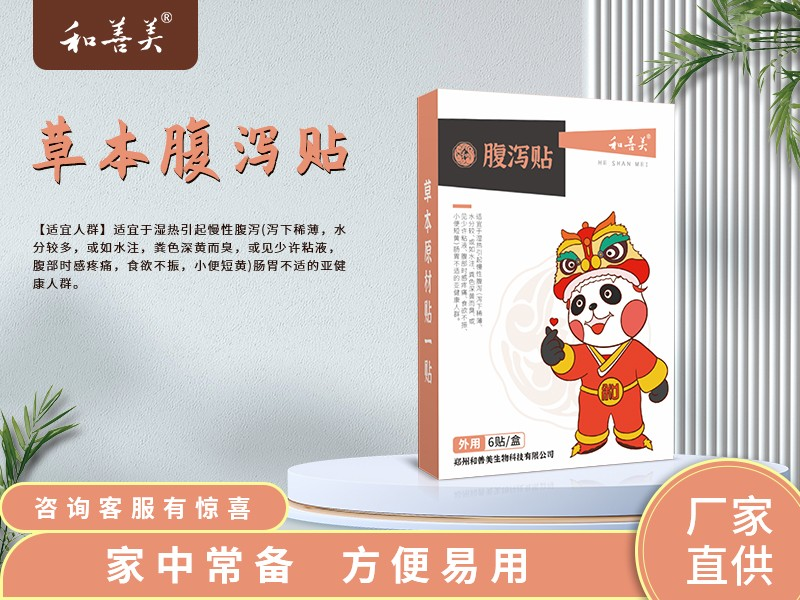腹泻贴
•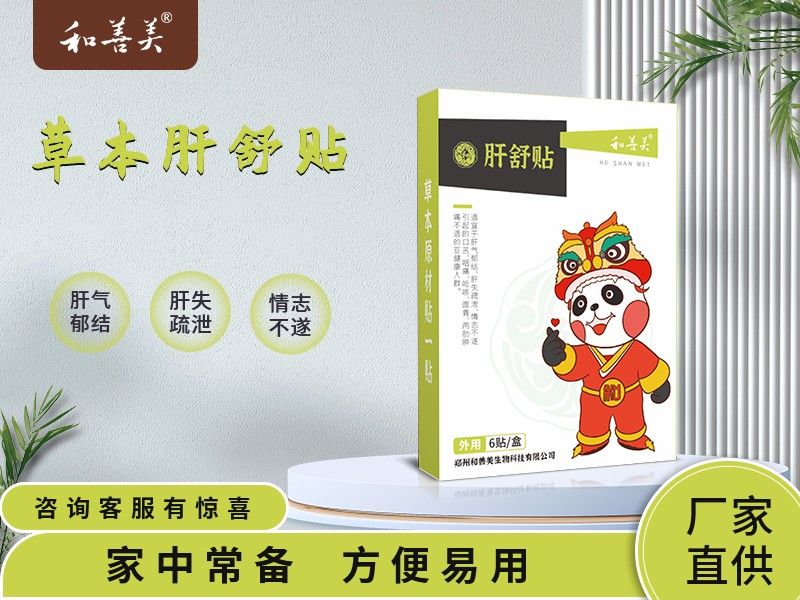肝舒贴
• 消毒产品
•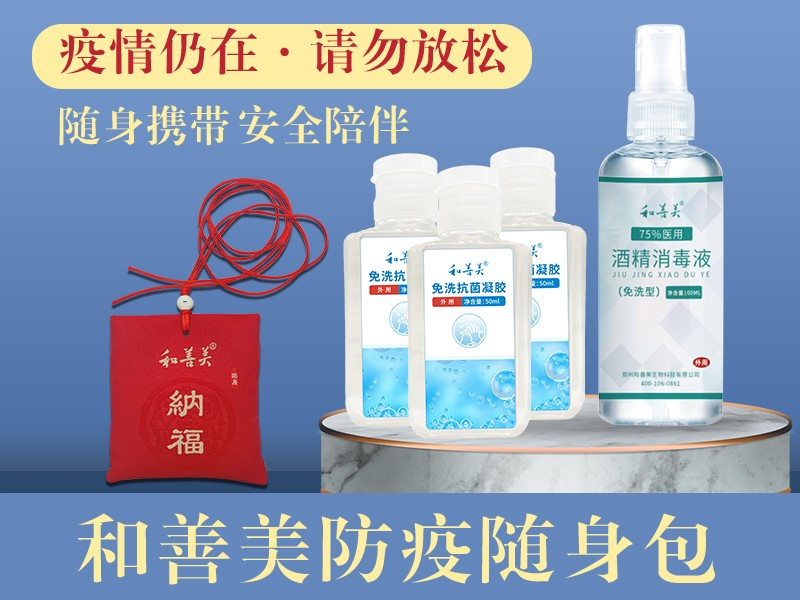防疫随身包
•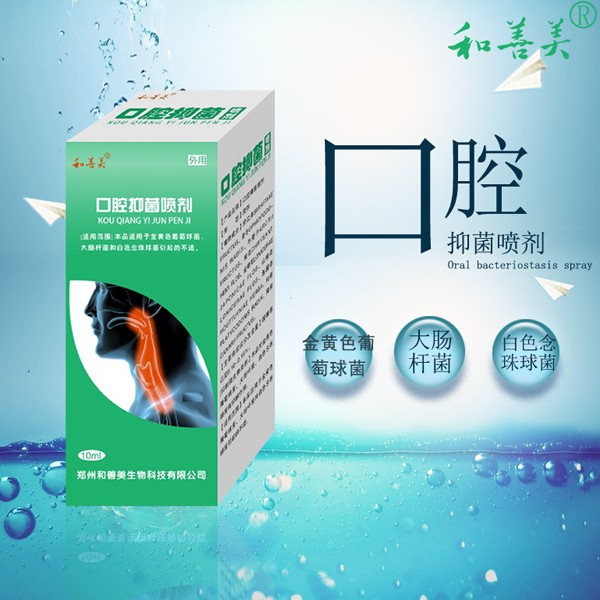口腔抑菌喷剂
•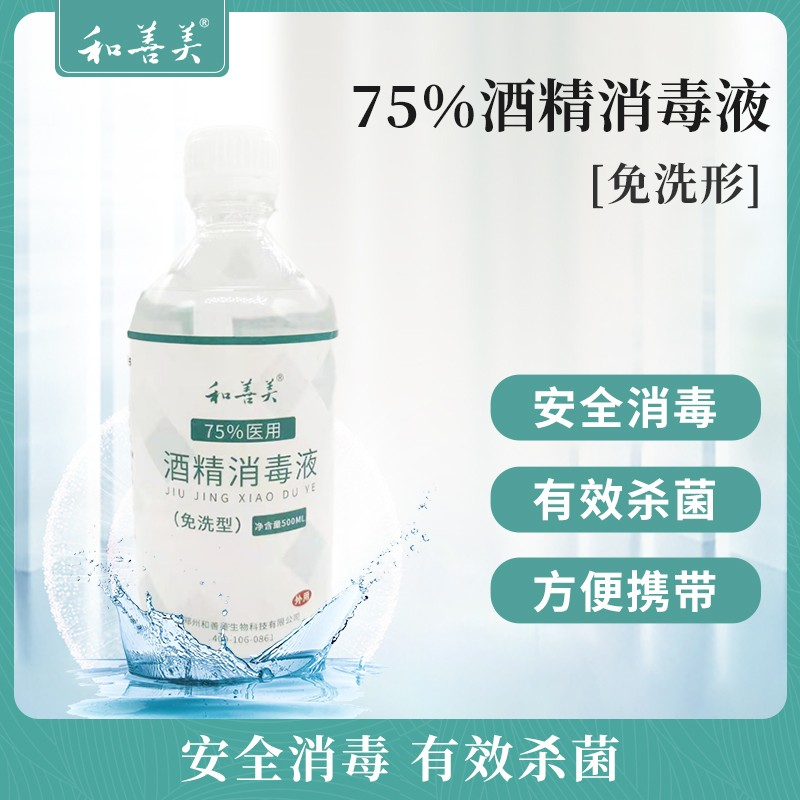75%医用酒精消毒液500ml
•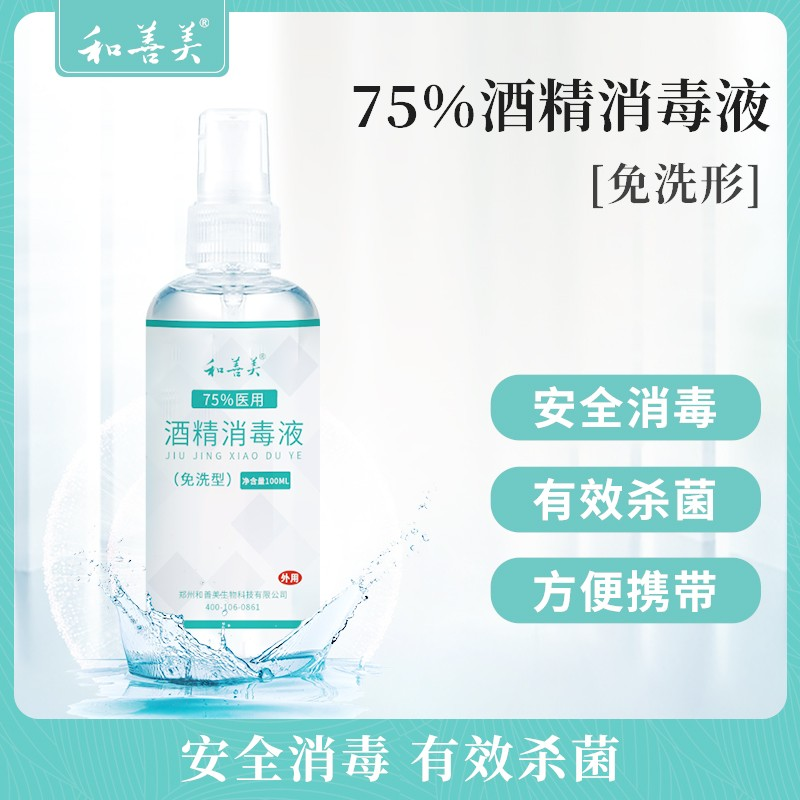75%医用酒精消毒液100ml
•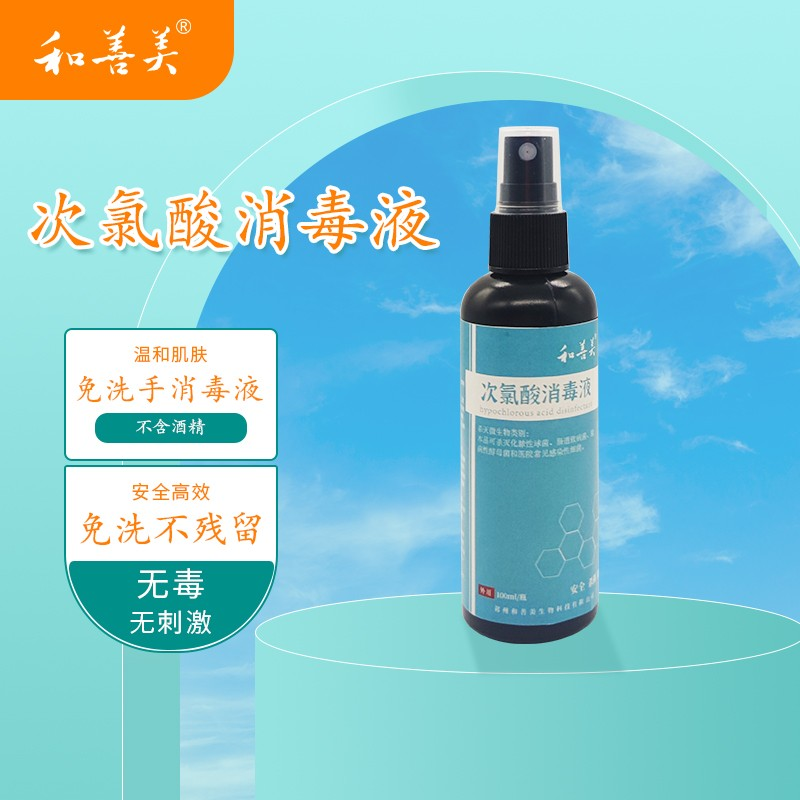次氯酸消毒液
•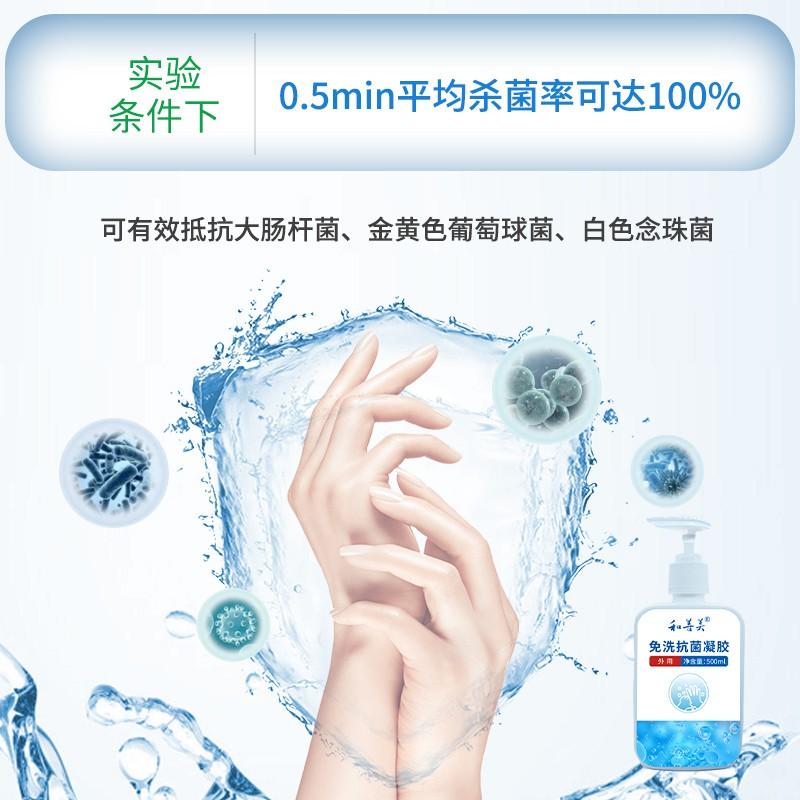抗菌免洗凝胶
• 眼贴
•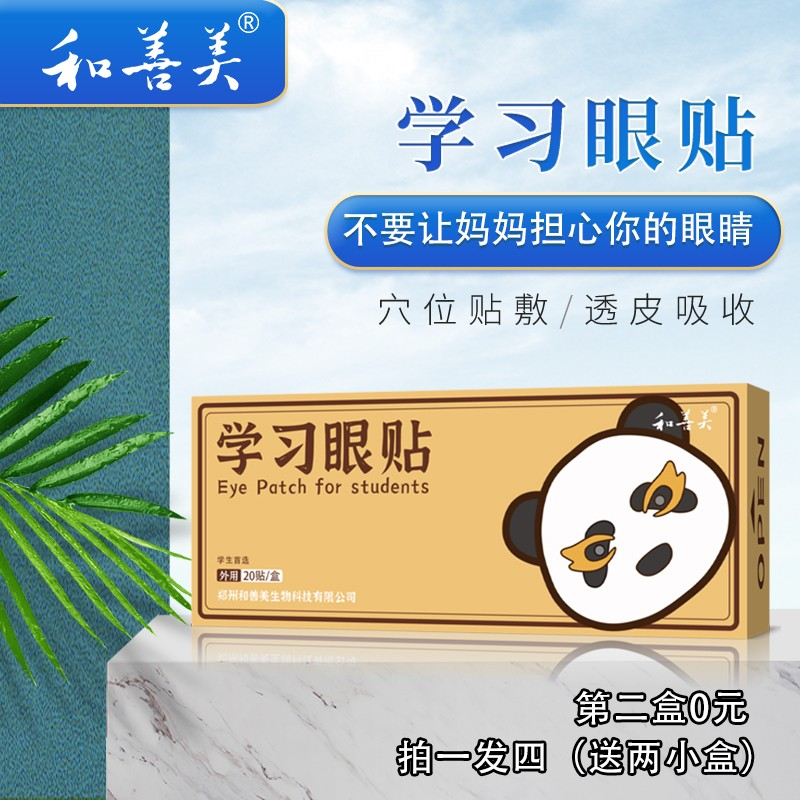学习眼贴
•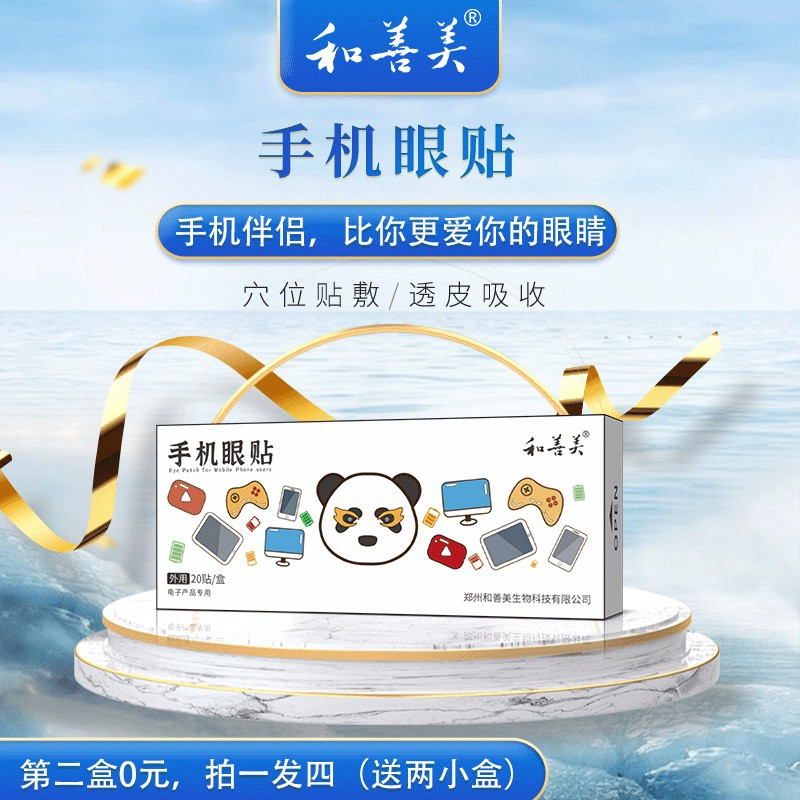手机眼贴
•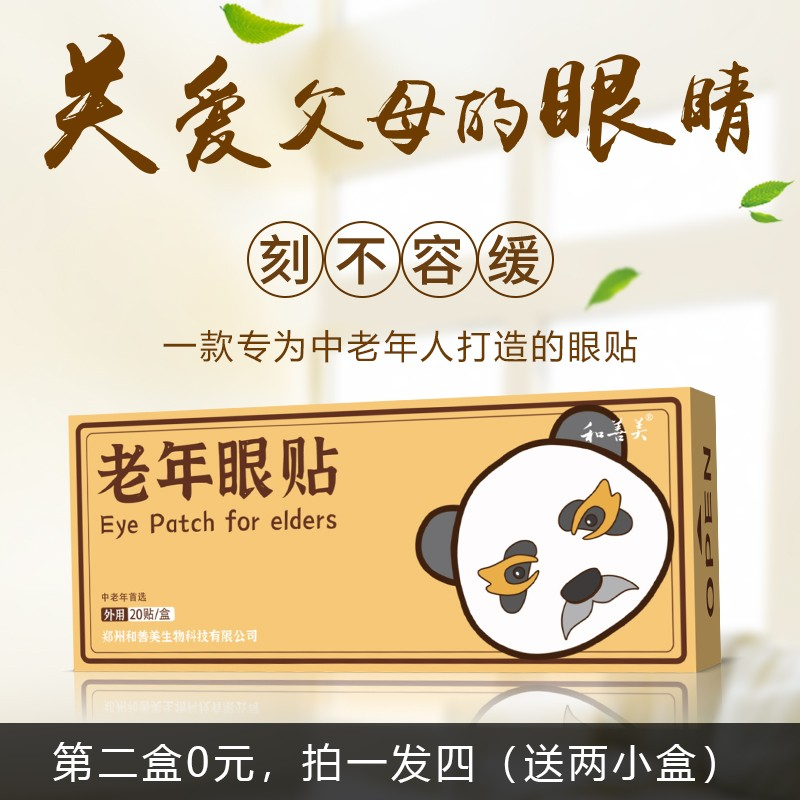老年眼贴
•司机眼贴
•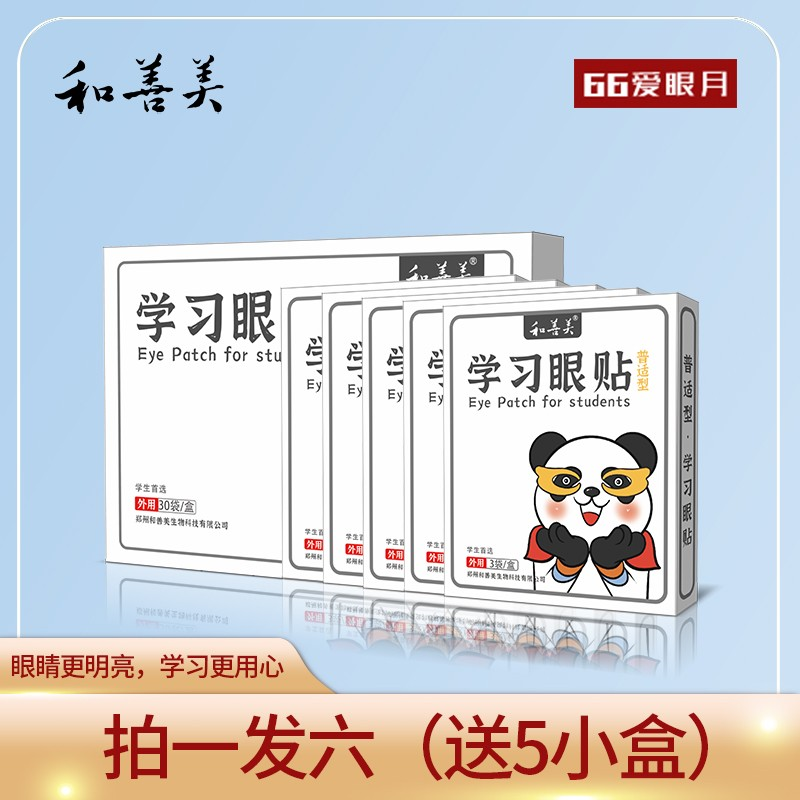学习眼贴(普适型)
•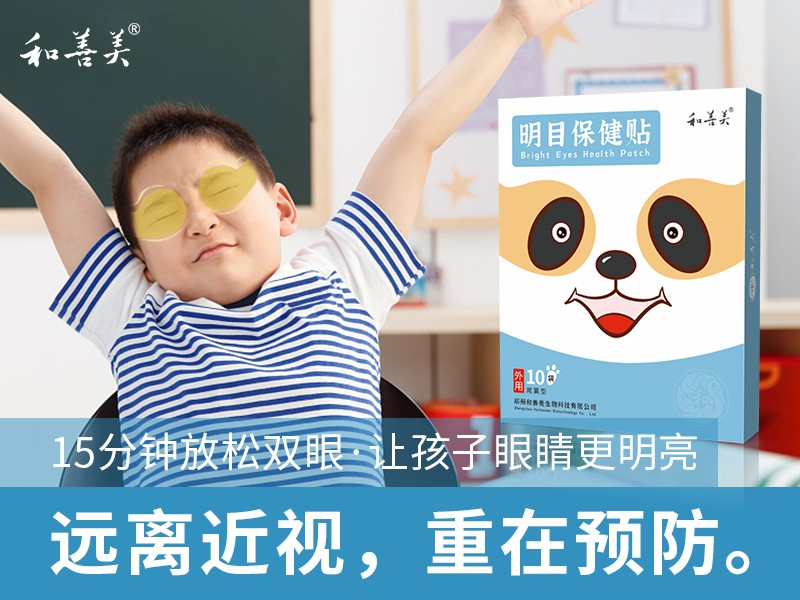明目保健贴（翼形）
•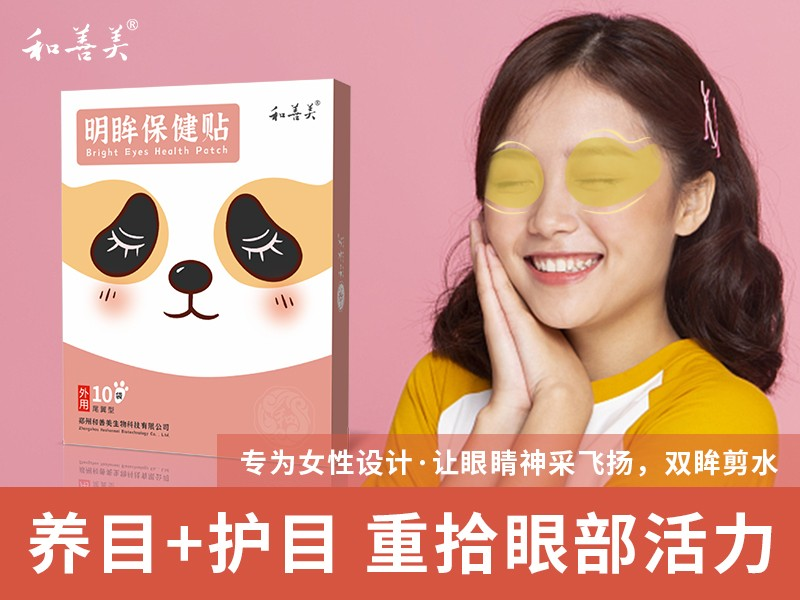明眸保健贴（翼形）
•护眼保健贴5袋装
•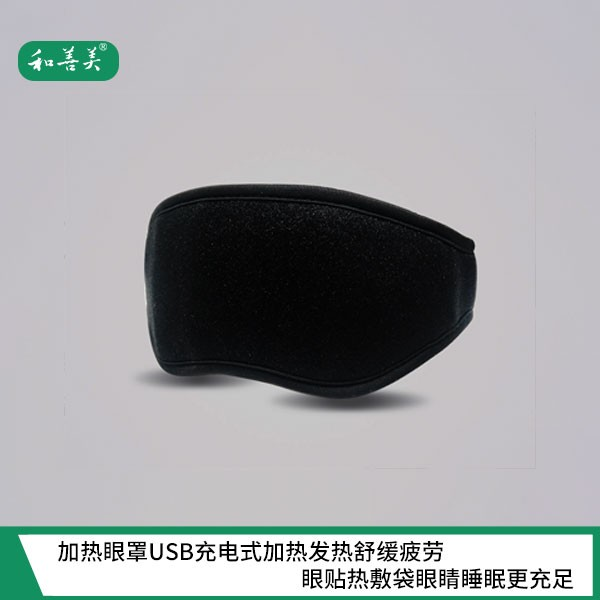加热眼罩
•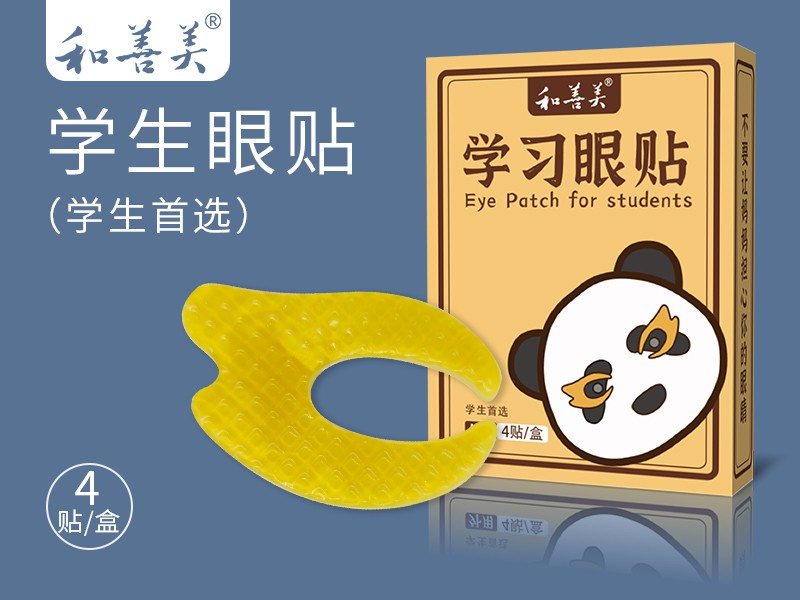学习眼贴
• 颈肩腰腿
•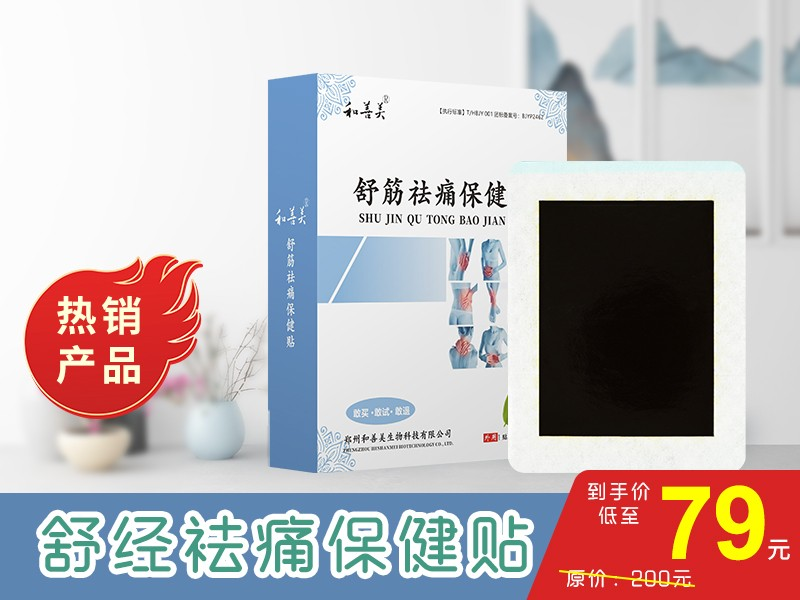舒筋祛痛小盒（10贴）
•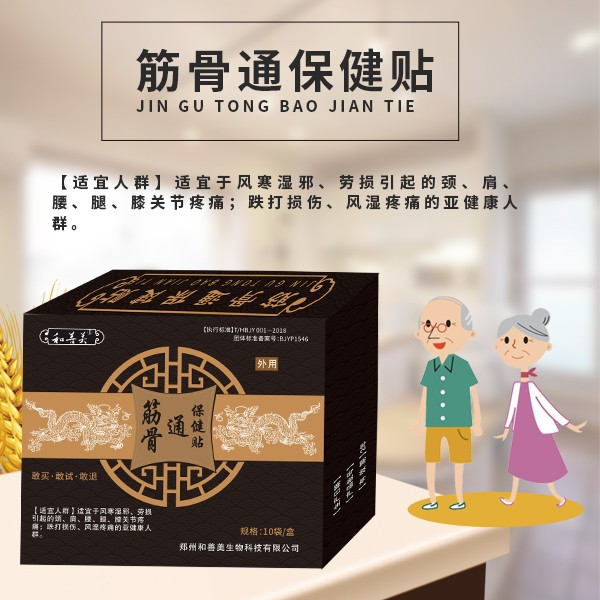筋骨通（小）保健贴
•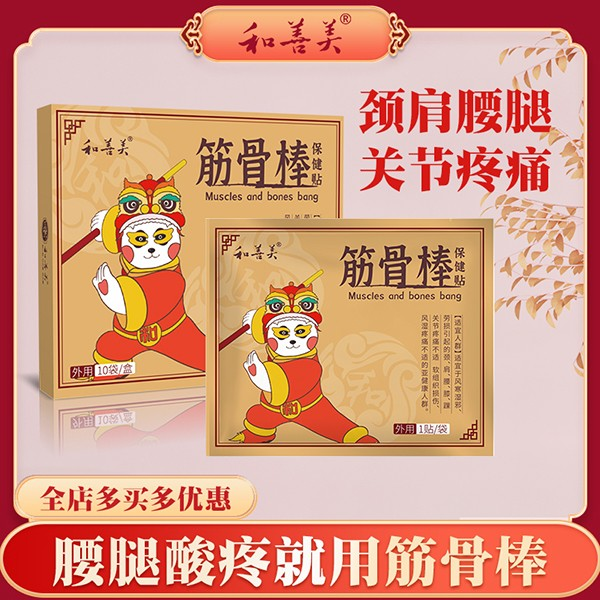筋骨棒保健贴
•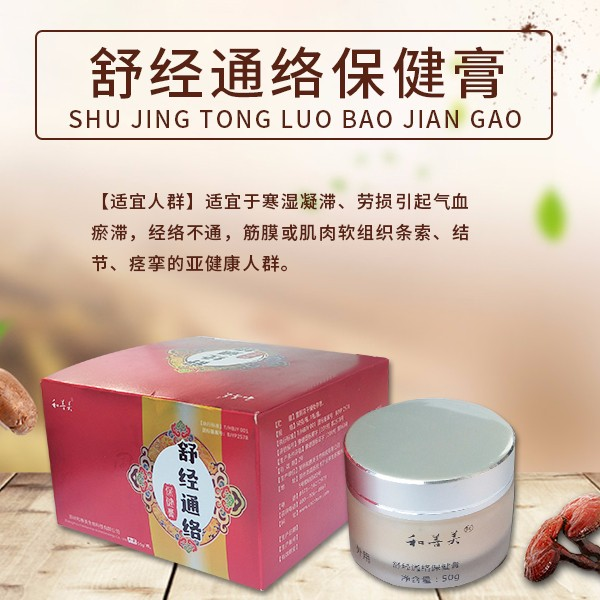舒经通络保健膏
•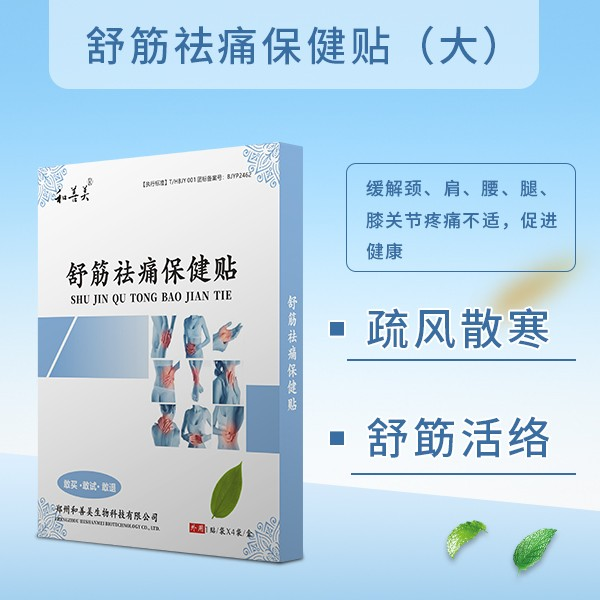舒筋祛痛大盒（4贴）
•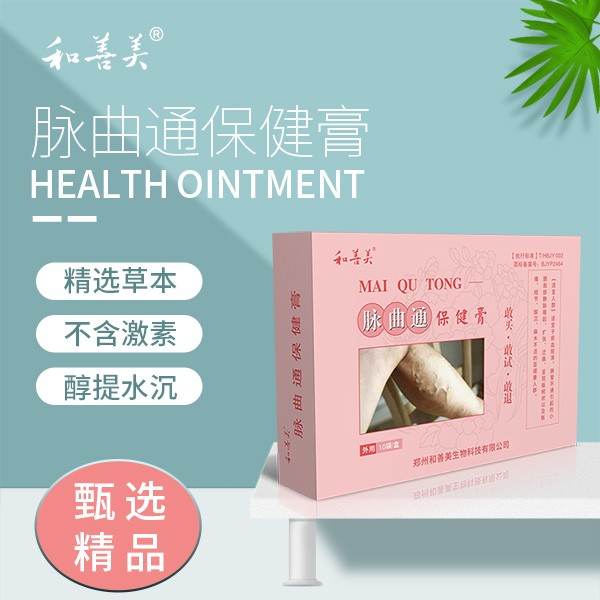脉曲通保健膏
•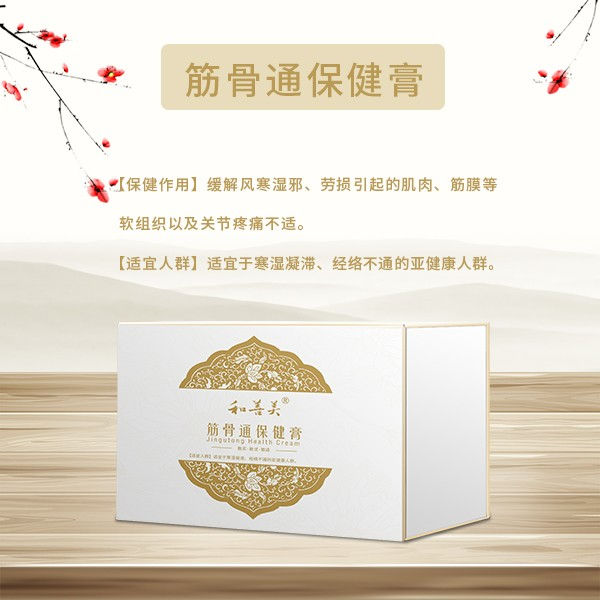筋骨通保健膏
•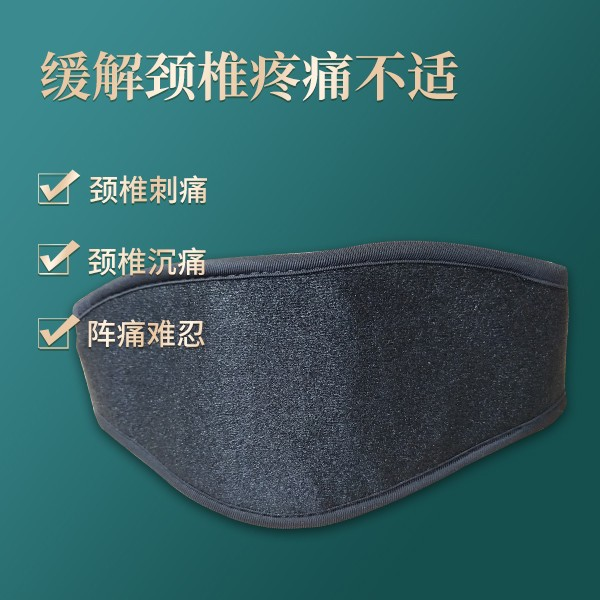颈椎辅助加热器
•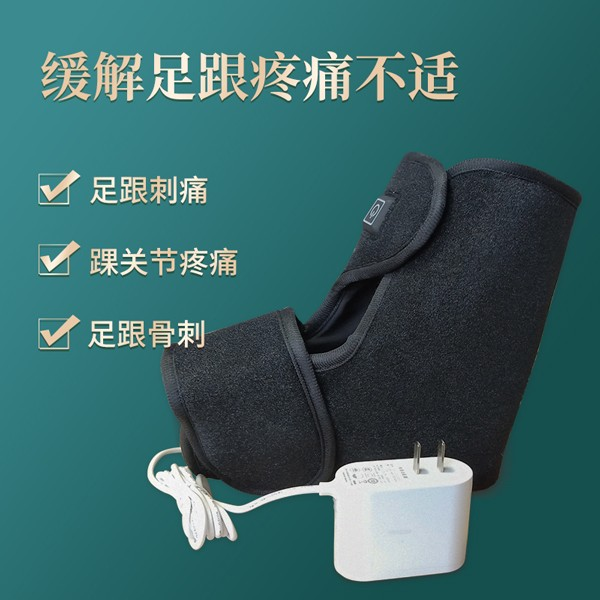足跟辅助加热器
•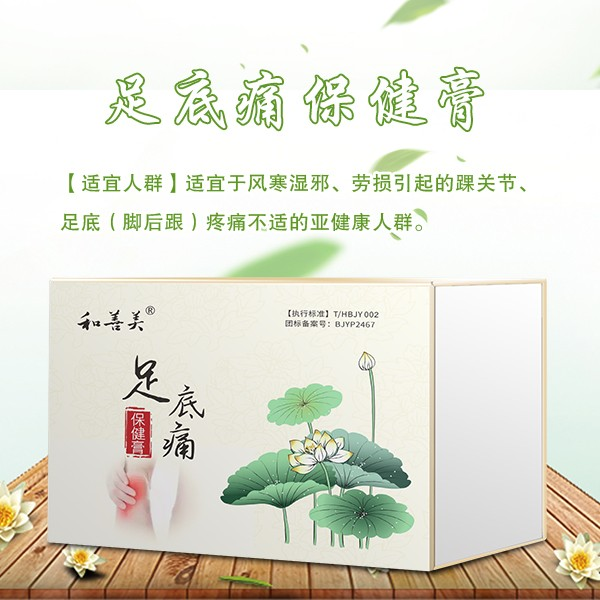足底痛保健膏
• 鼻贴
•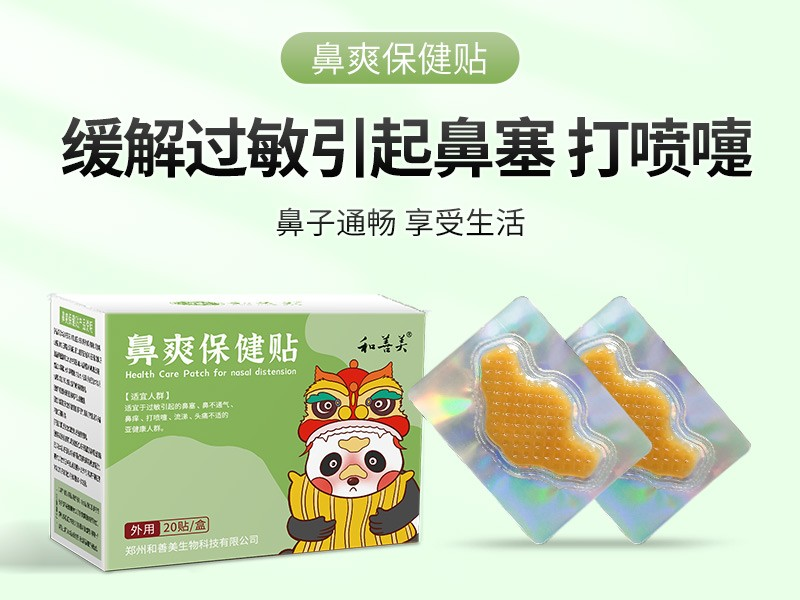鼻爽保健贴
•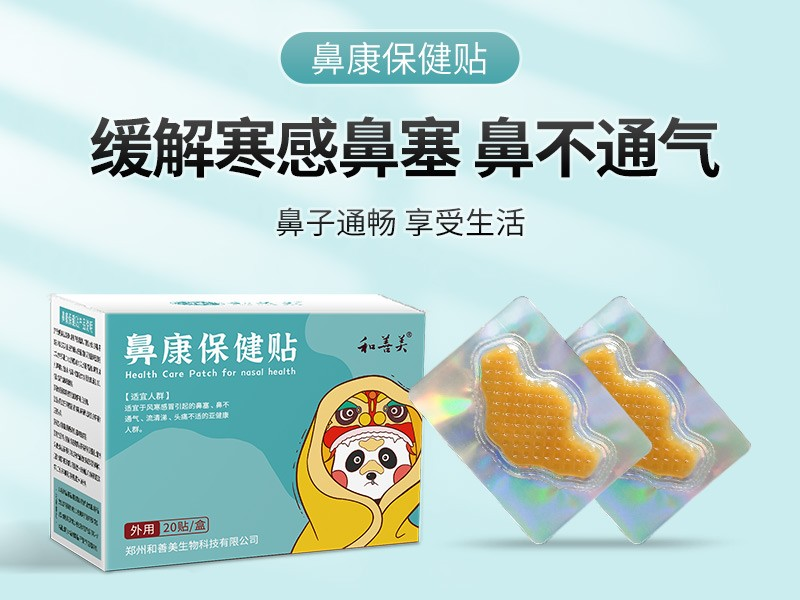鼻康保健贴
•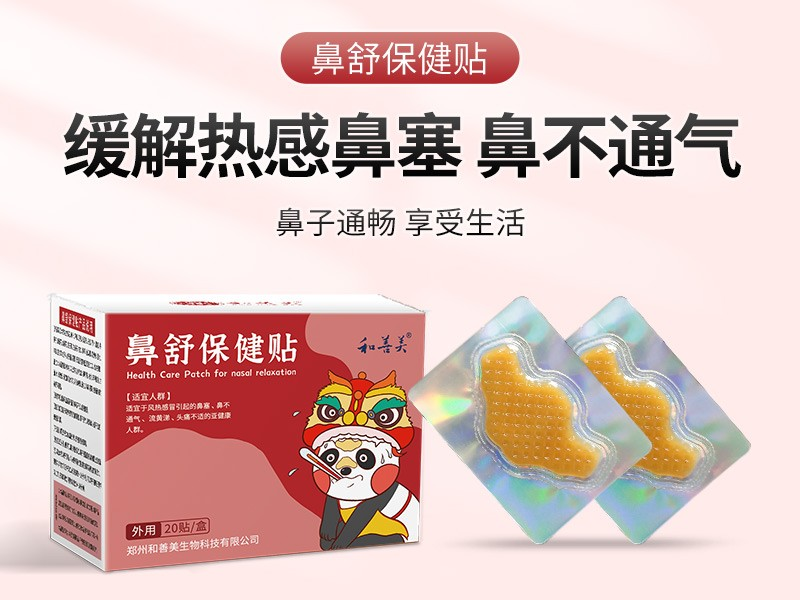鼻舒保健贴
• 祛痘美白
• 保健膏
•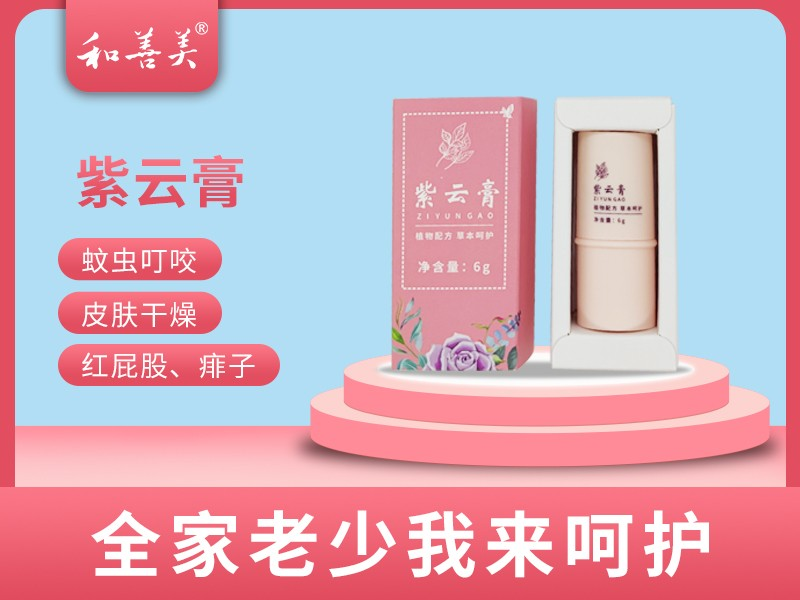紫云膏
•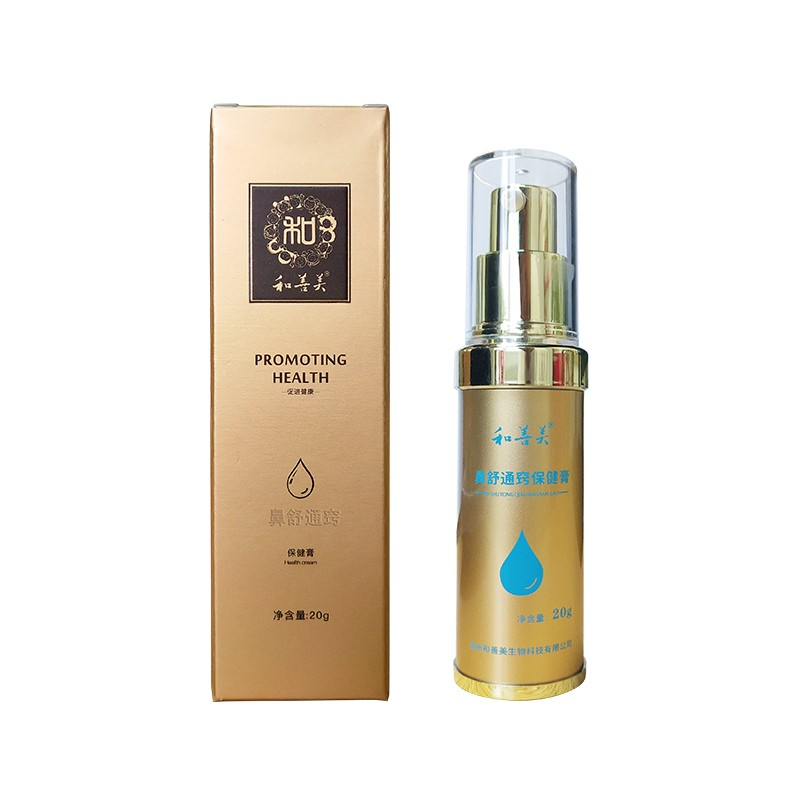鼻舒通窍保健膏
•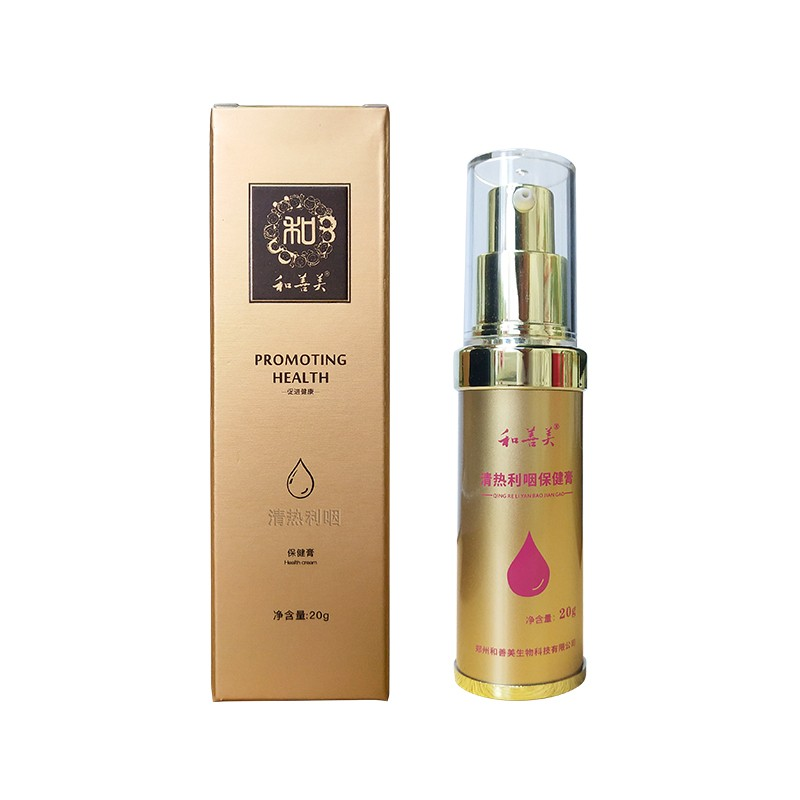清热利咽保健膏
•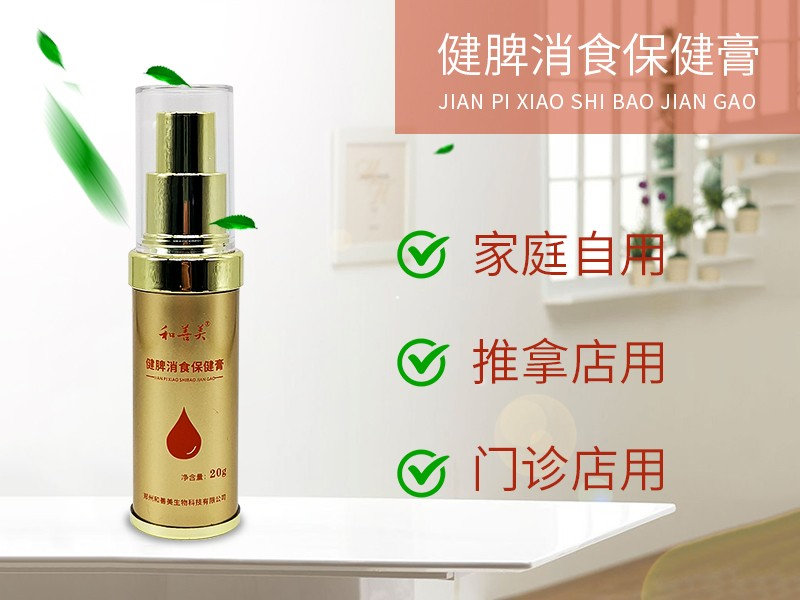健脾消食保健膏
•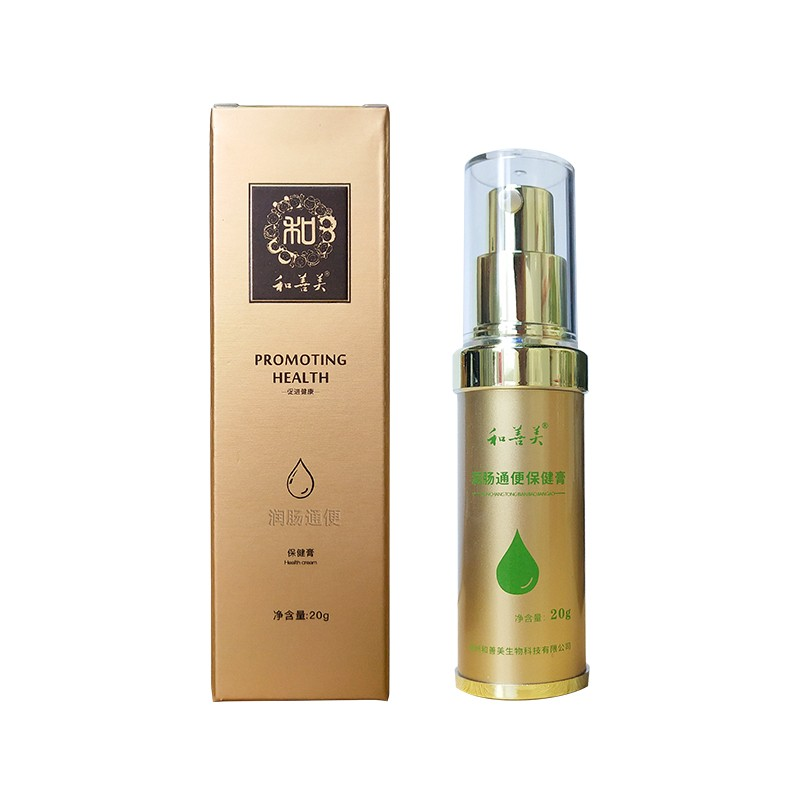润肠通便保健膏
•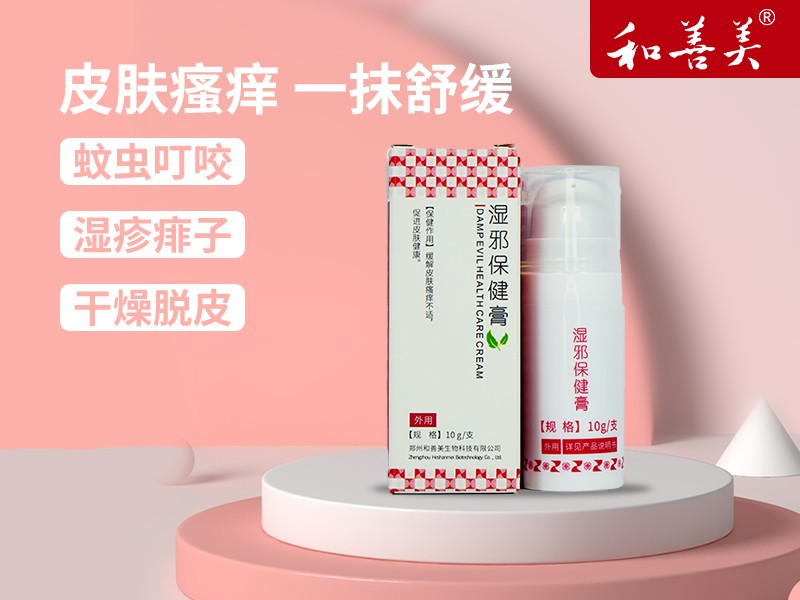湿邪保健膏
•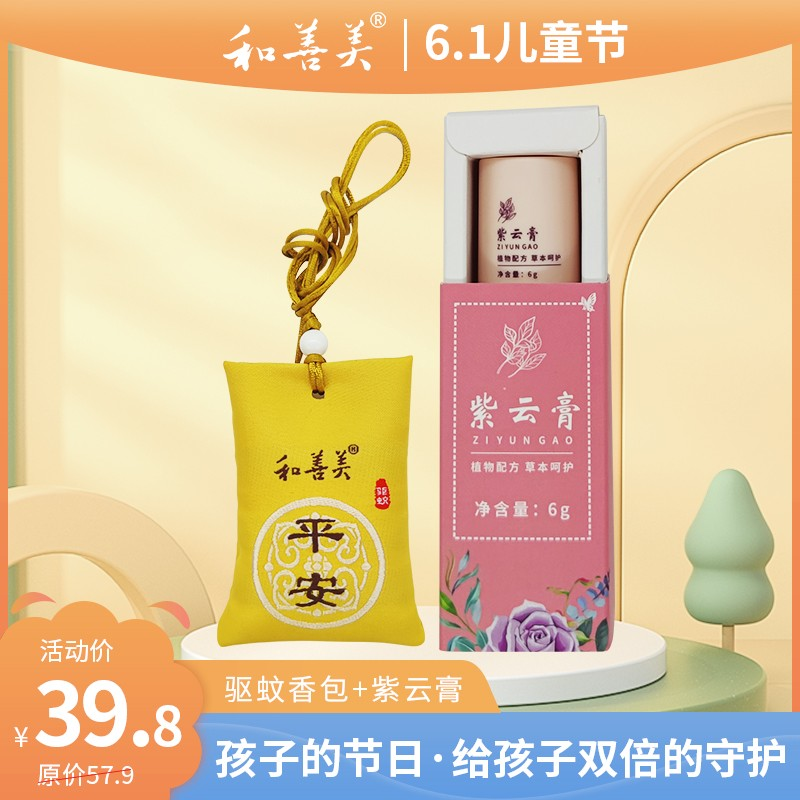紫云膏驱蚊香包
•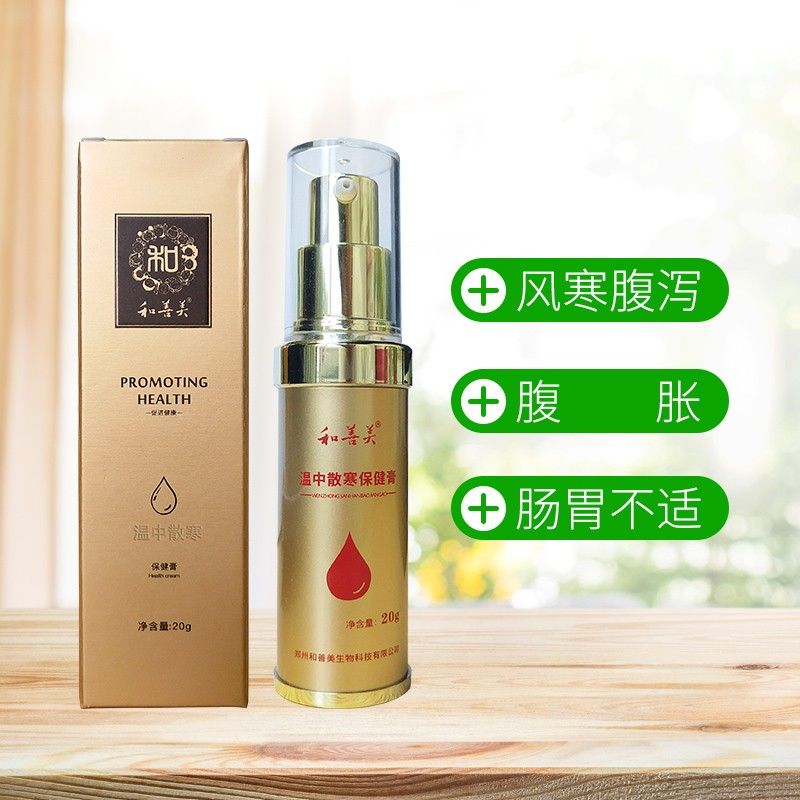温中散寒保健膏
•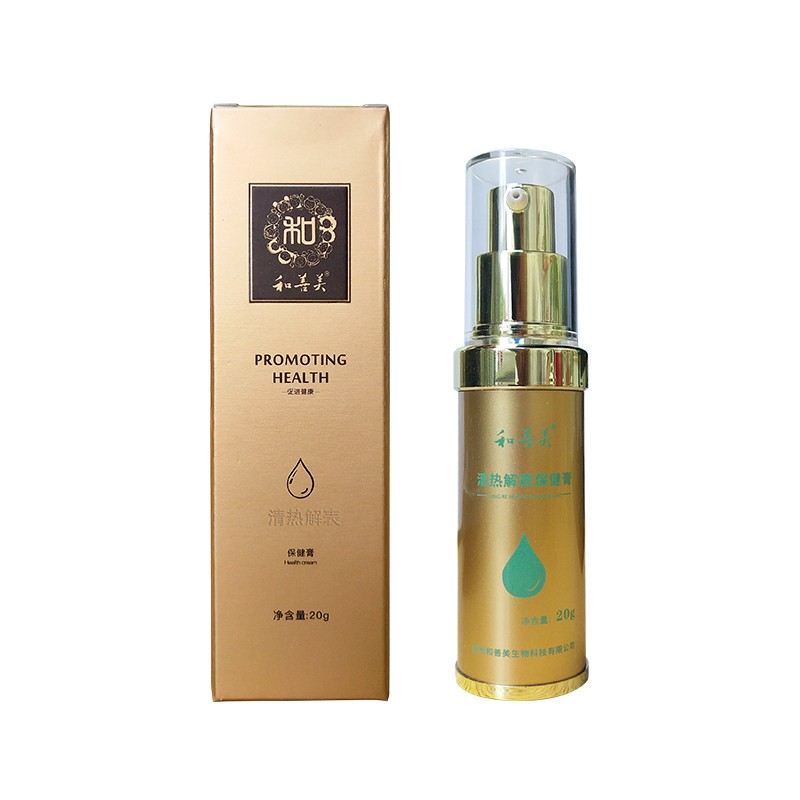清热解表保健膏
•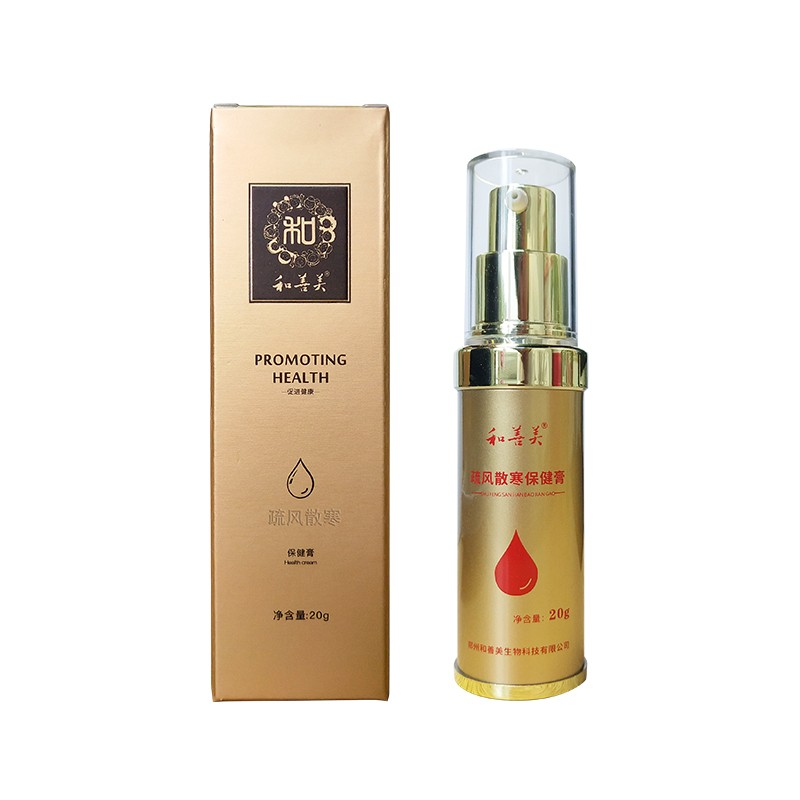疏风散寒保健膏
•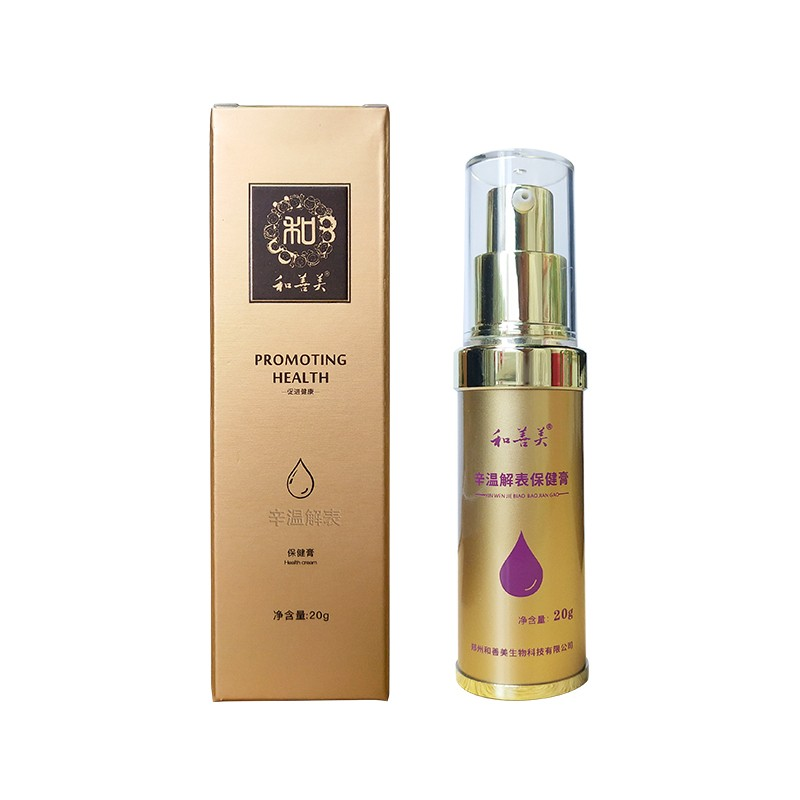辛温解表保健膏
• 保健粉
•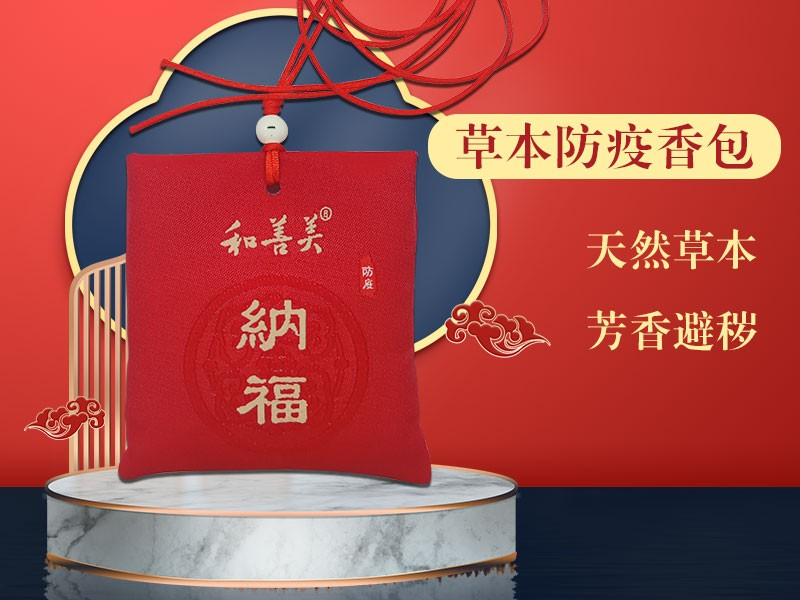防疫香包
•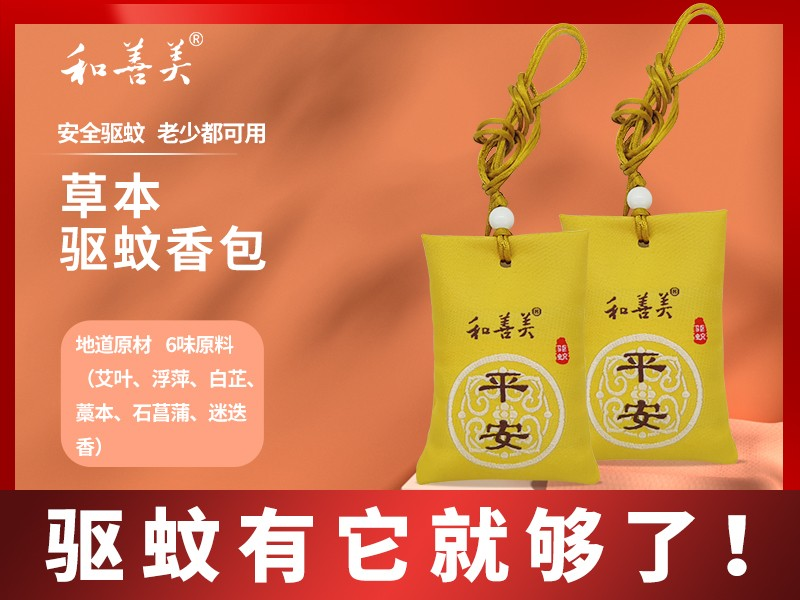驱蚊香包
•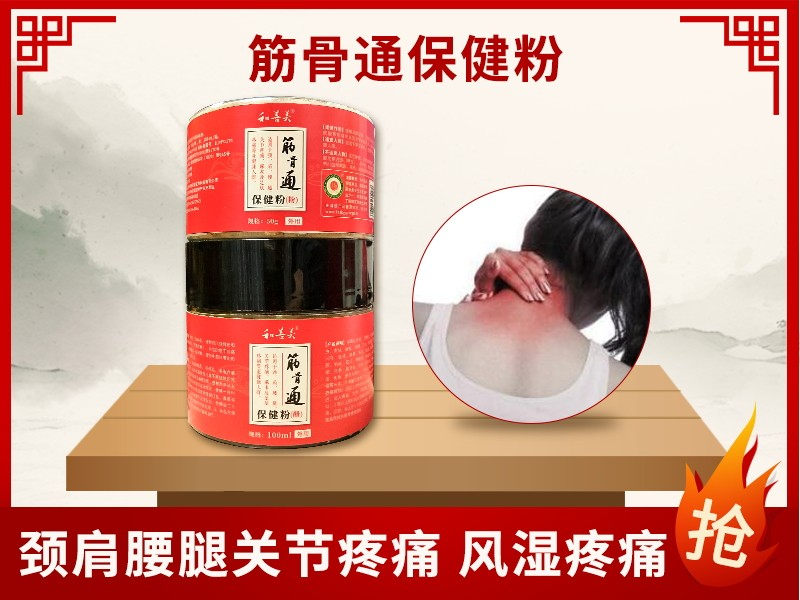筋骨通保健粉
•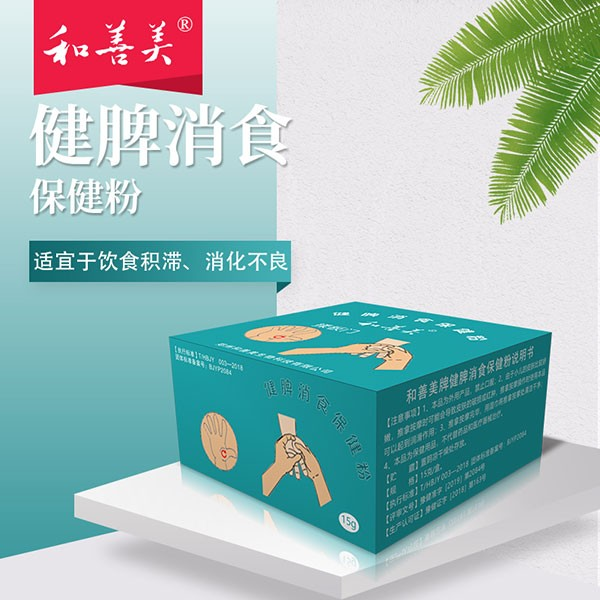健脾消食保健粉
•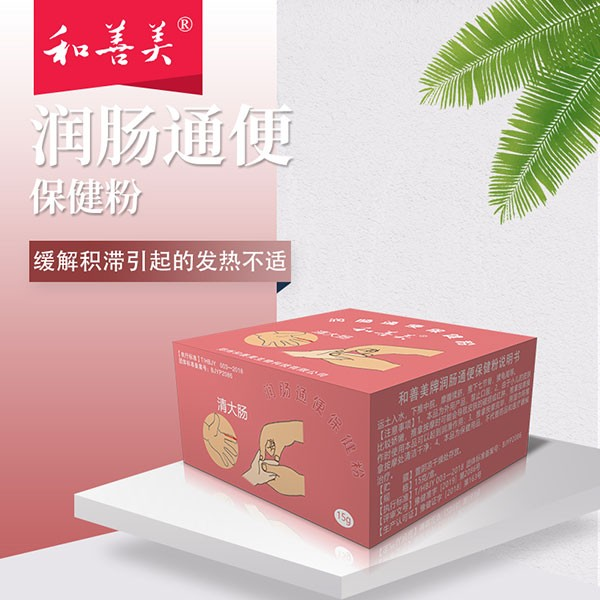润肠通便保健粉
•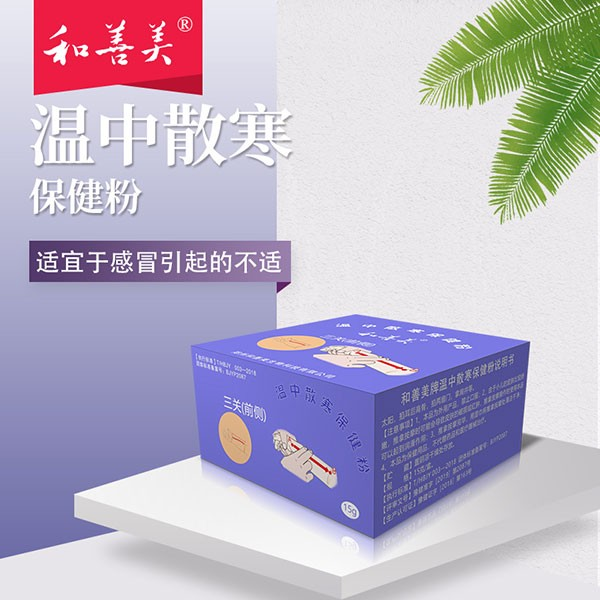温中散寒保健粉
•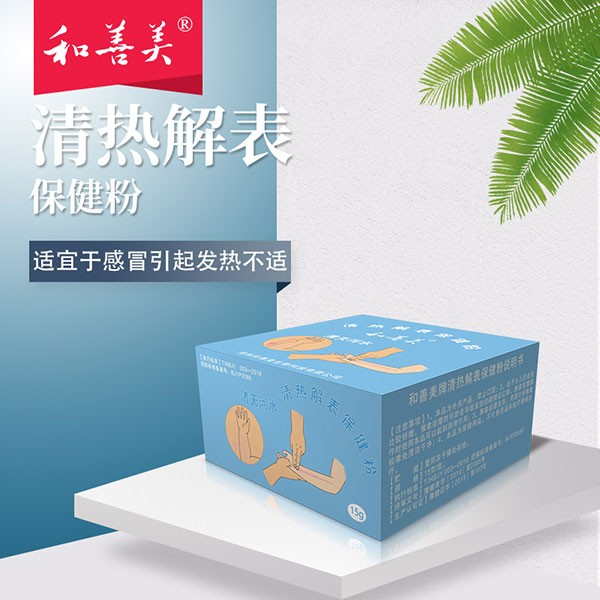清热解表保健粉
• 艾宁贴
• 护牙系列
•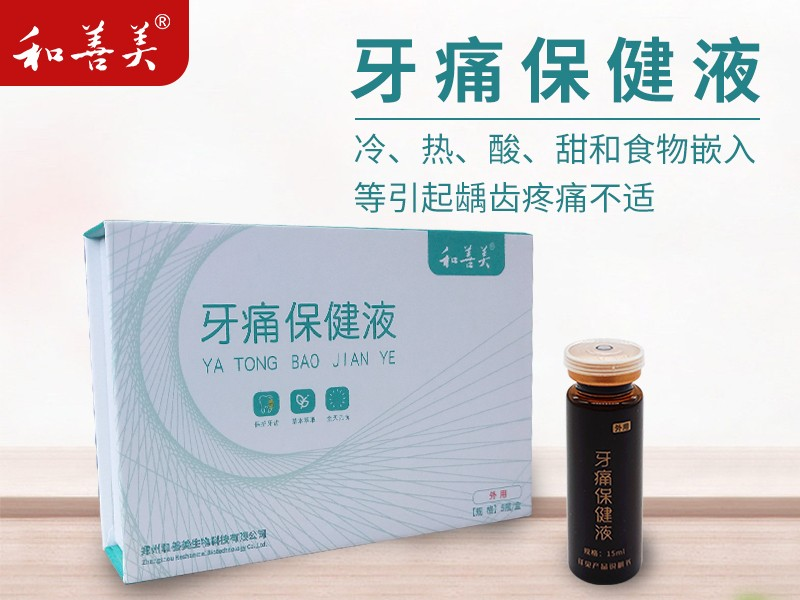牙痛保健液
•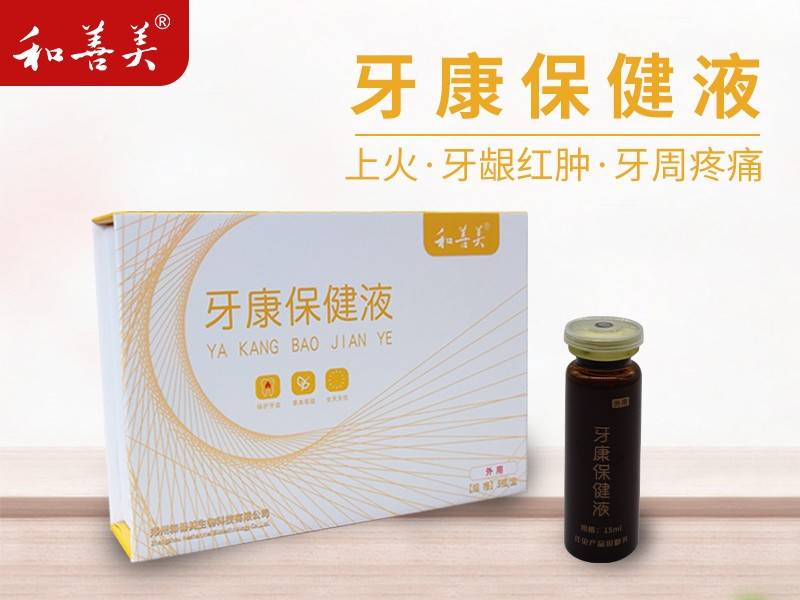牙康保健液
• 女性产品
•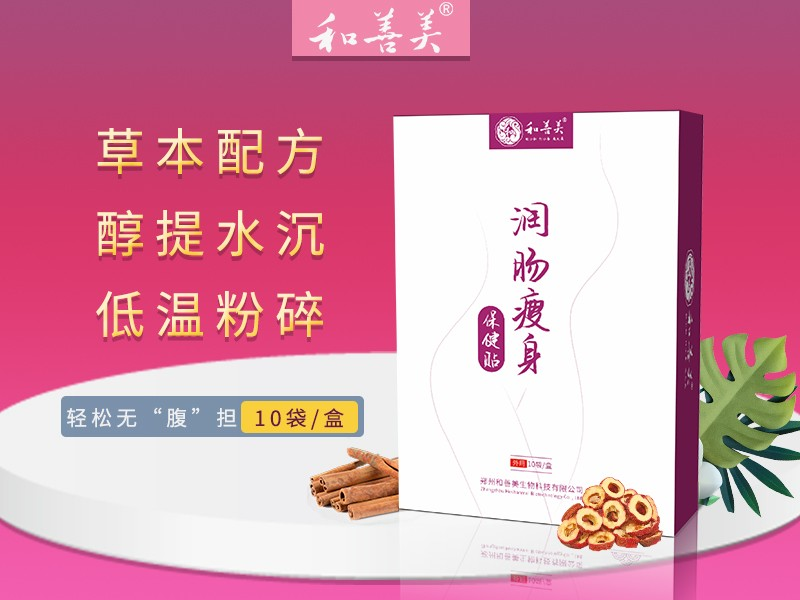润肠瘦身保健贴
•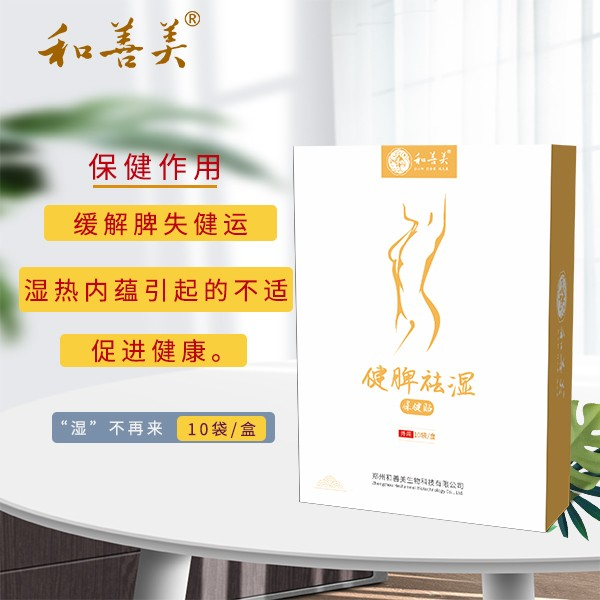健脾祛湿保健贴
•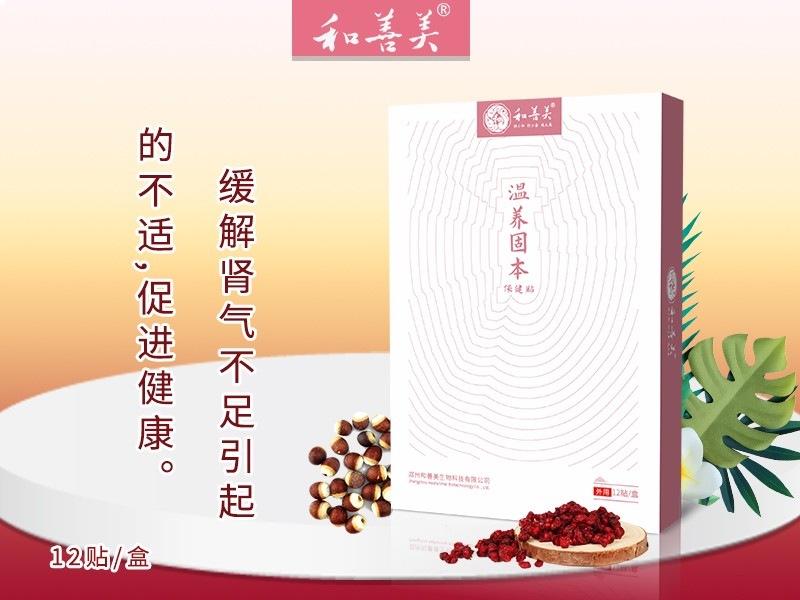温养固本保健贴
• 男性产品
•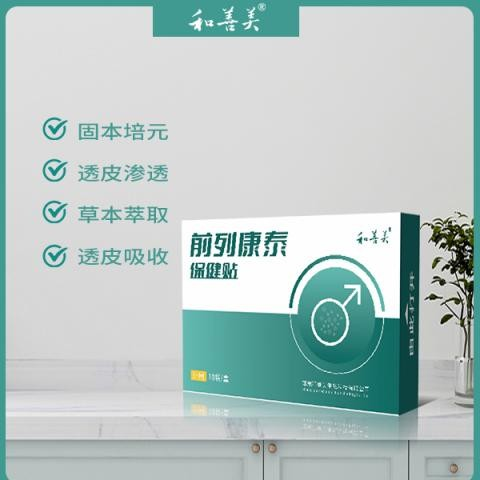前列康泰保健贴
•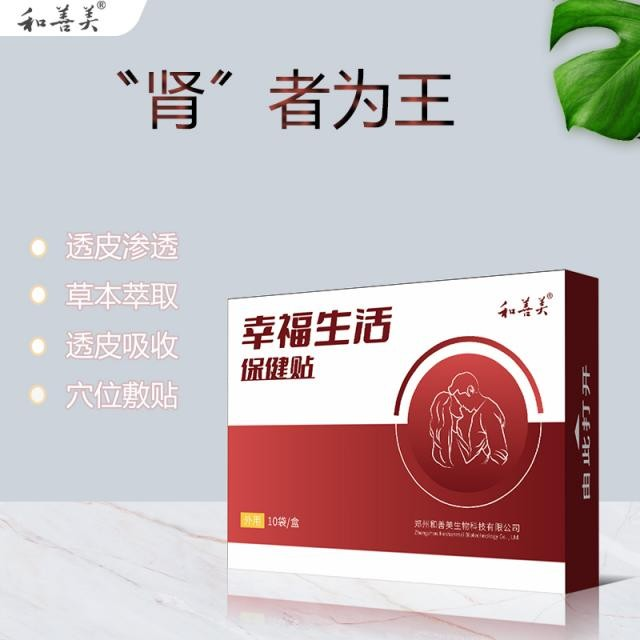幸福生活保健贴
•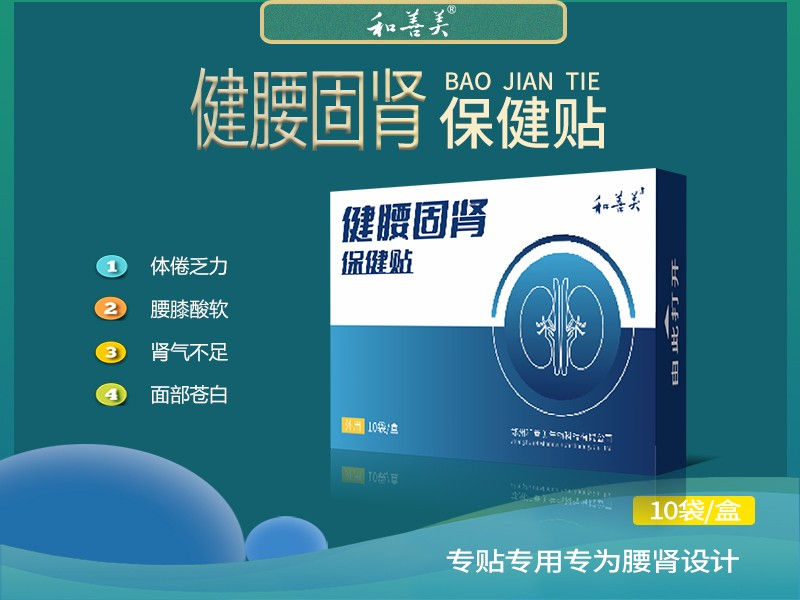健腰固肾保健贴

1、会员注册流程：打开手机微信，搜索健康和善美公众号，点击关注公众号，点击申请页面，选择需要申请的客户级别、输入个人姓名、手机号，验证码等信息，输入完毕后，查看申请协议，无异议后，点击已阅读按钮并提交即可完成注册。

• 您还没有收藏商品